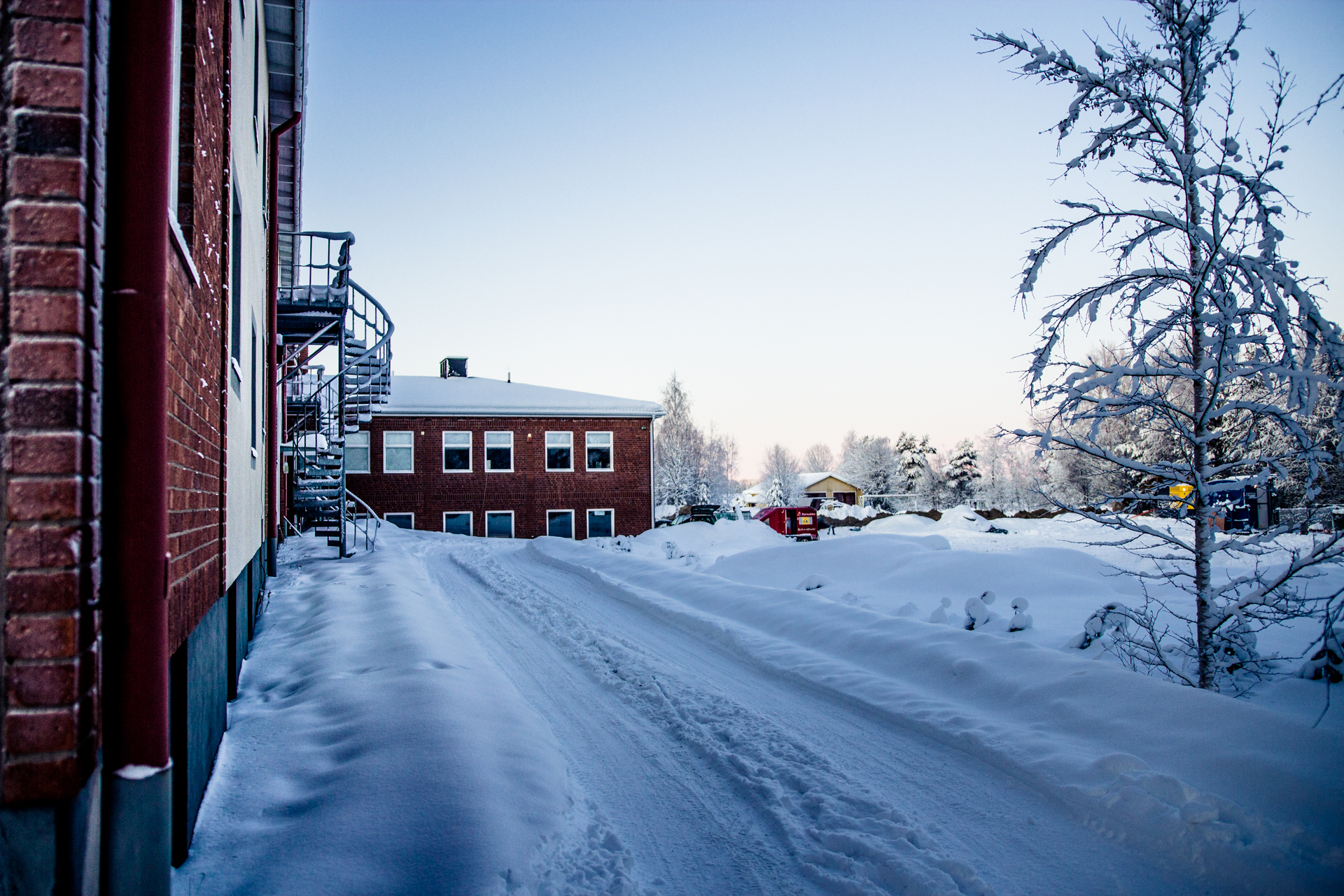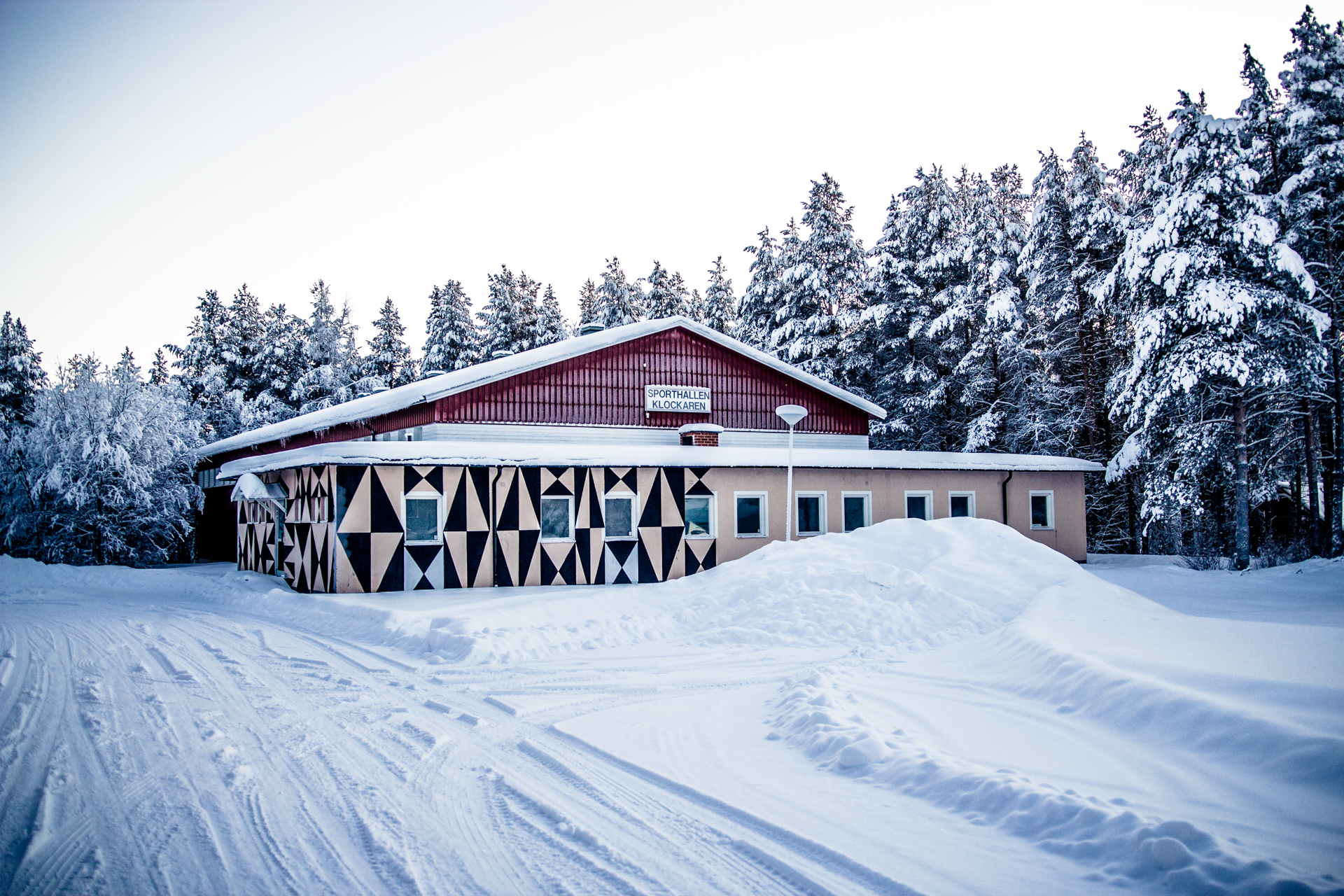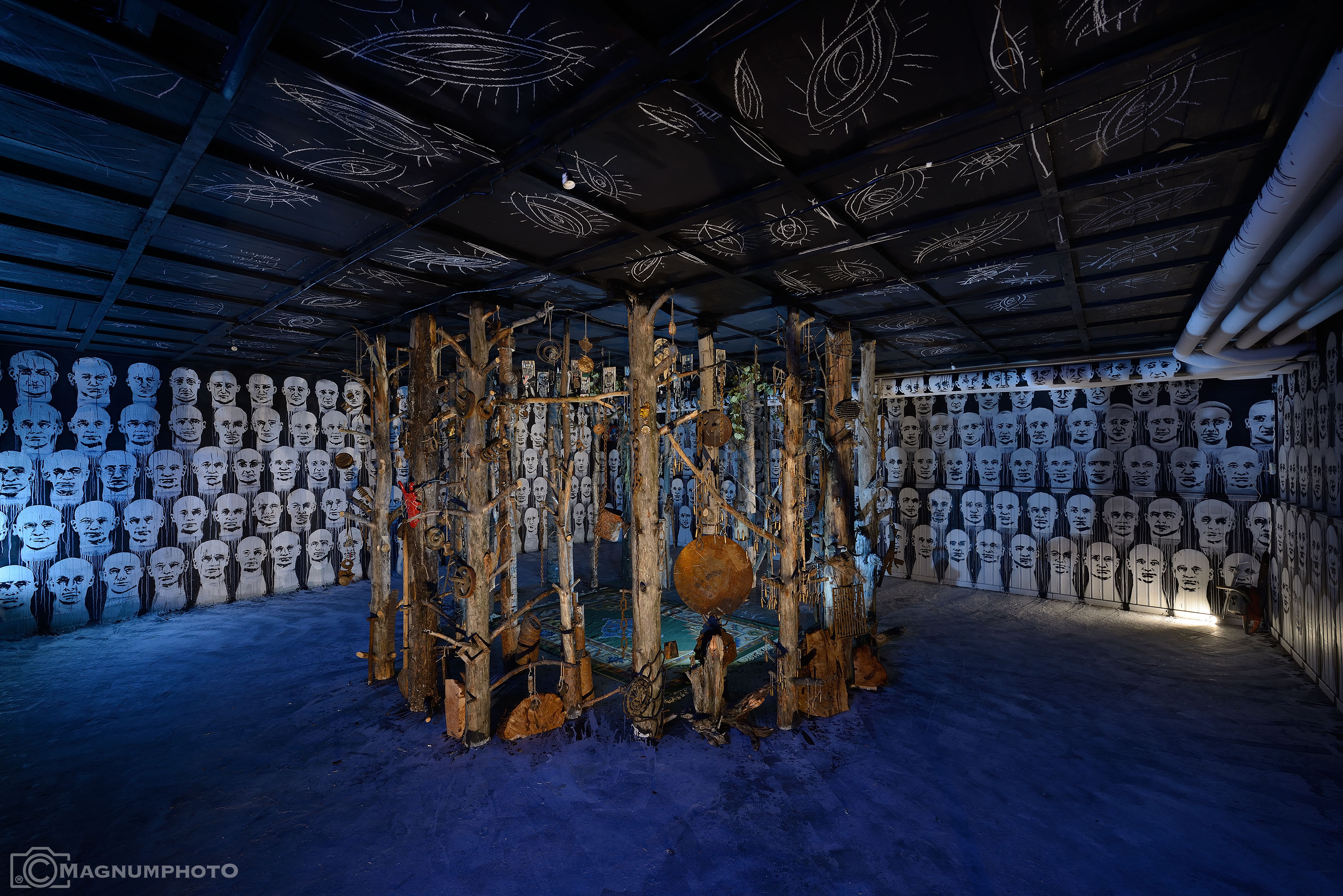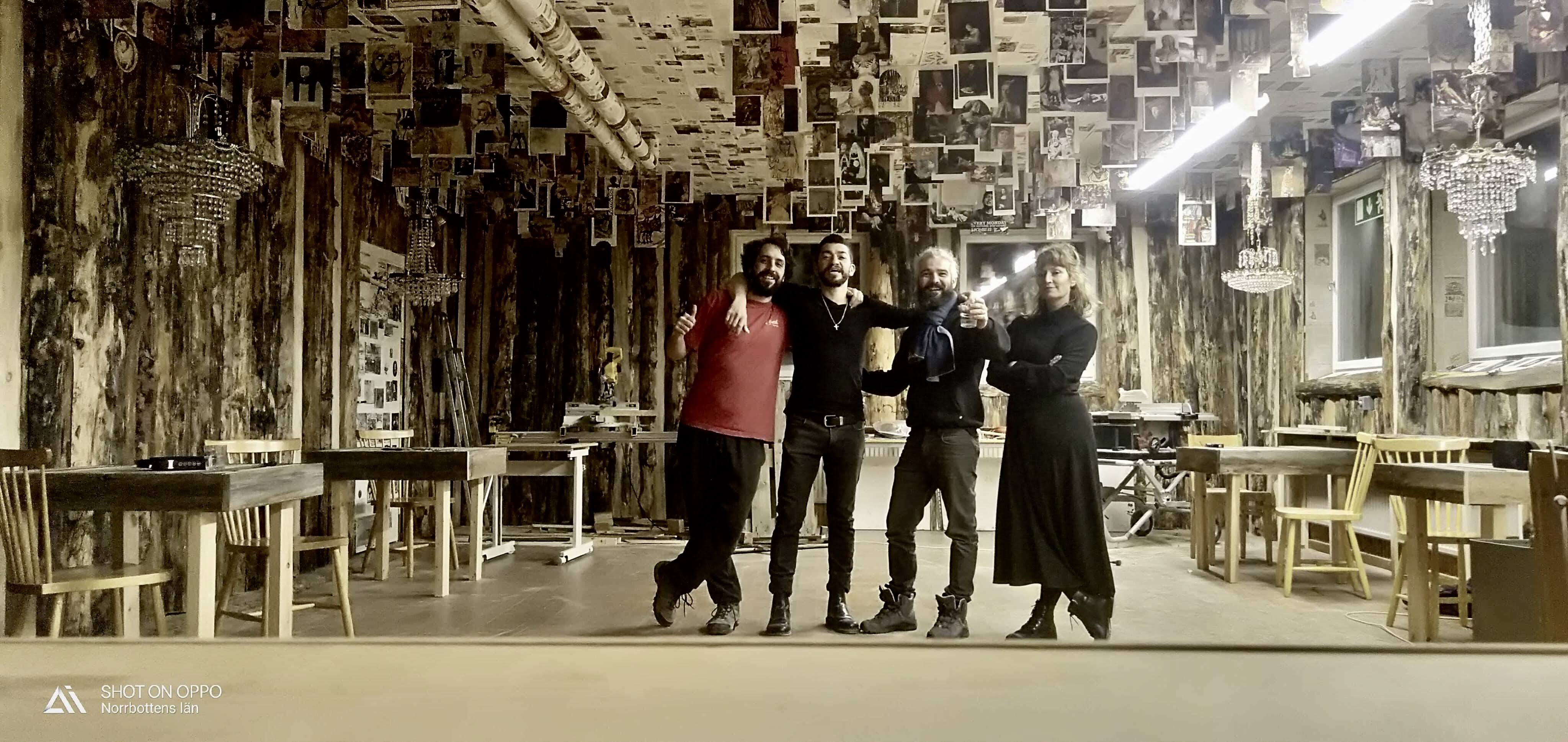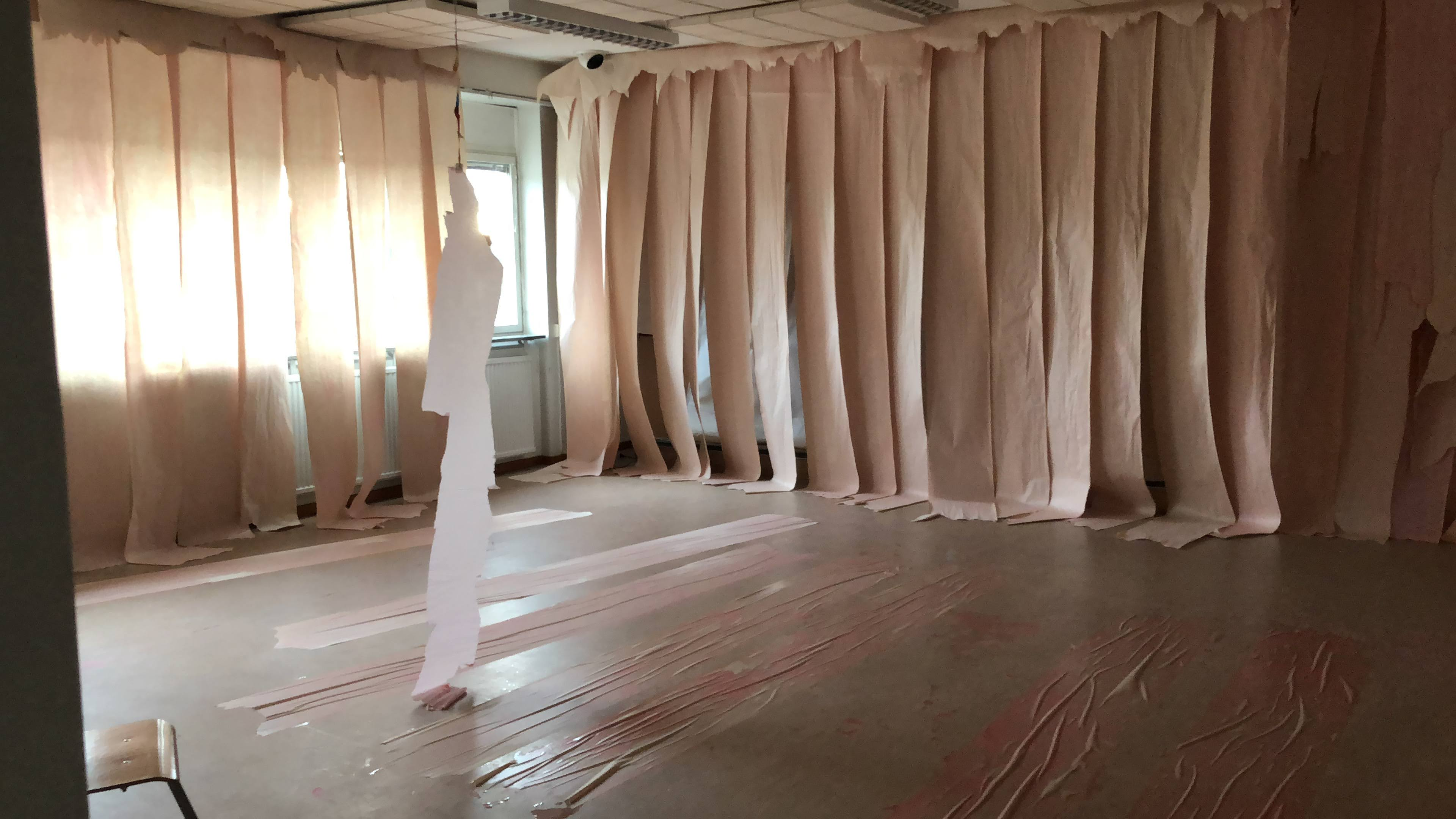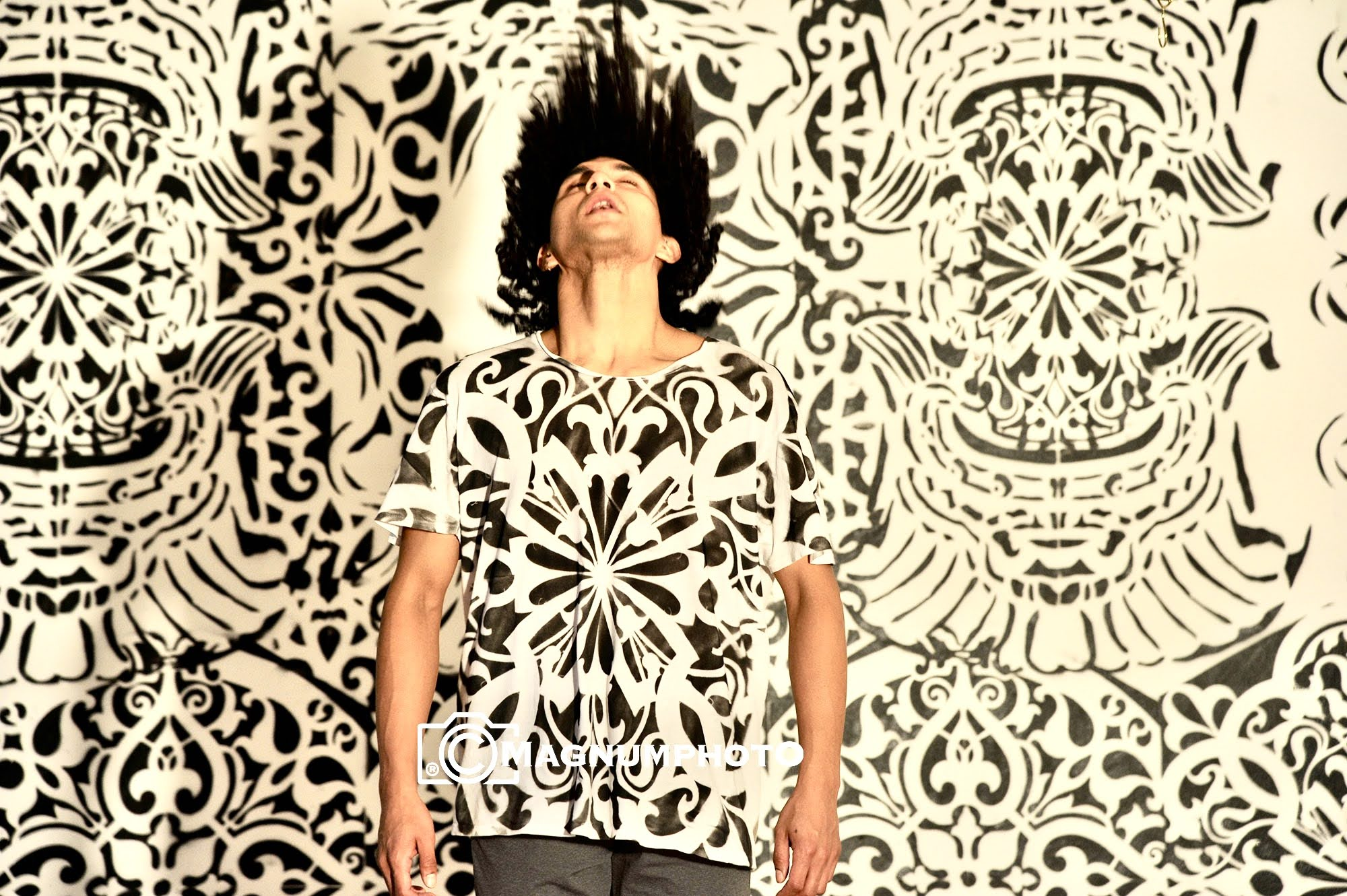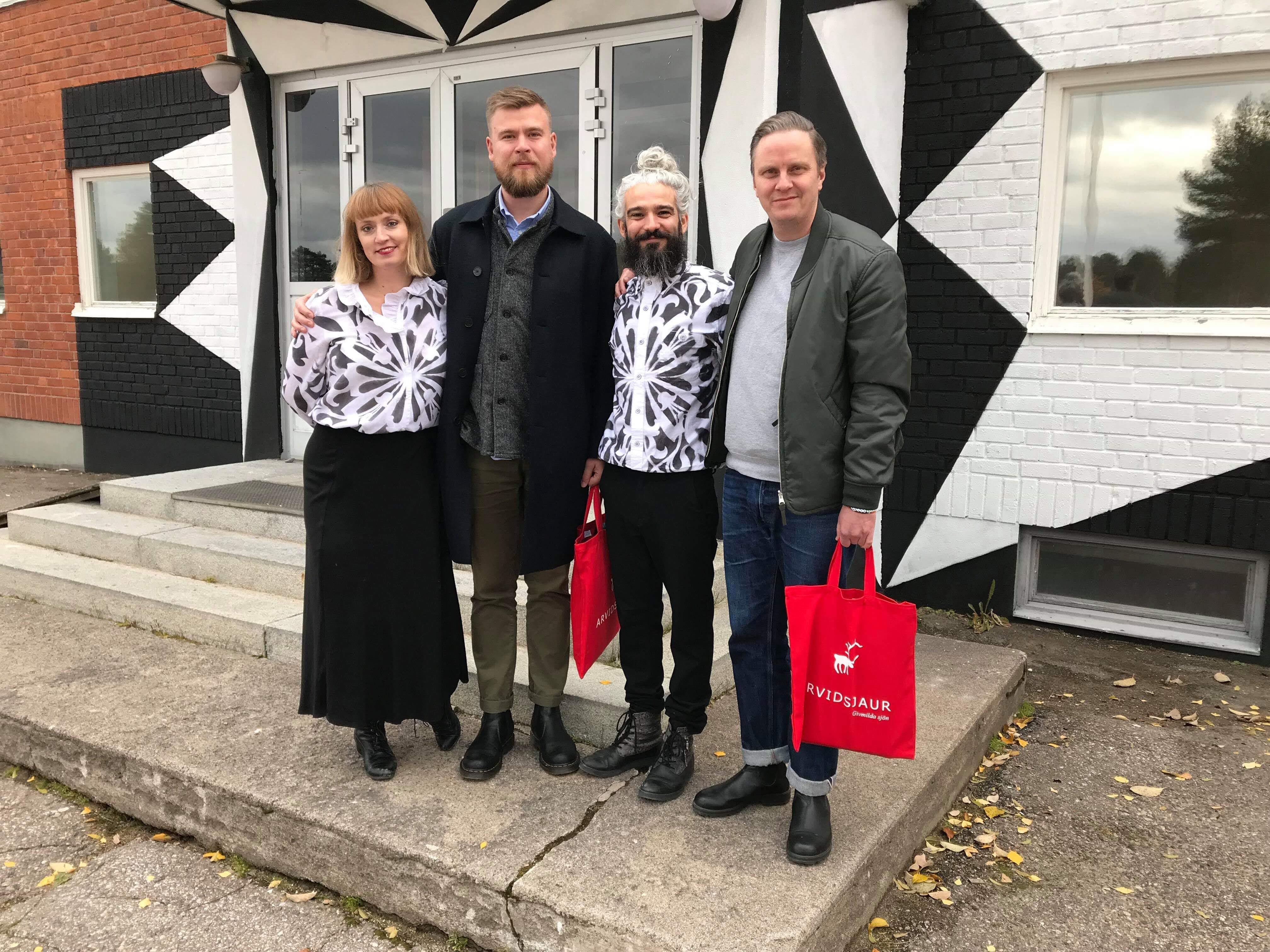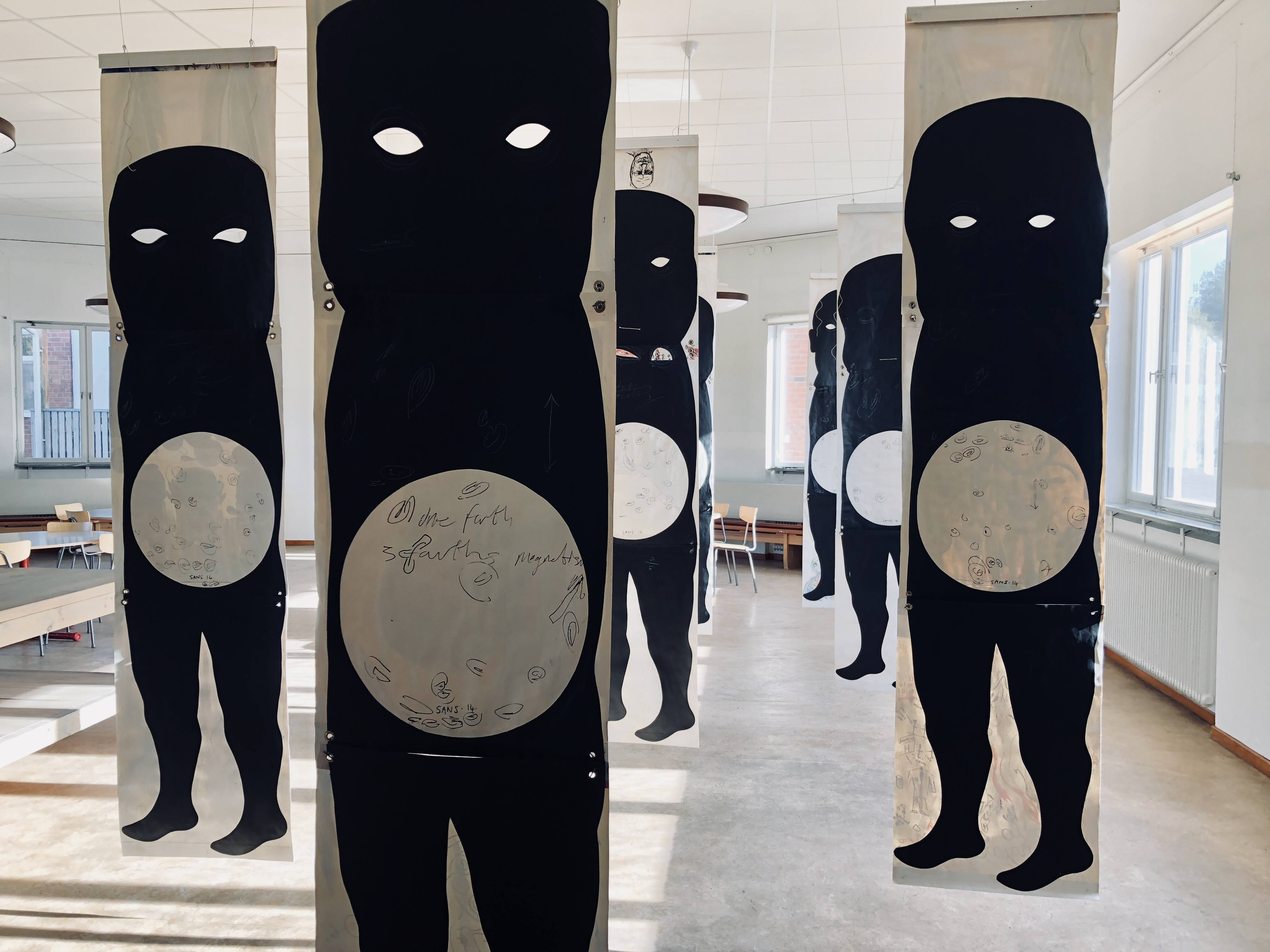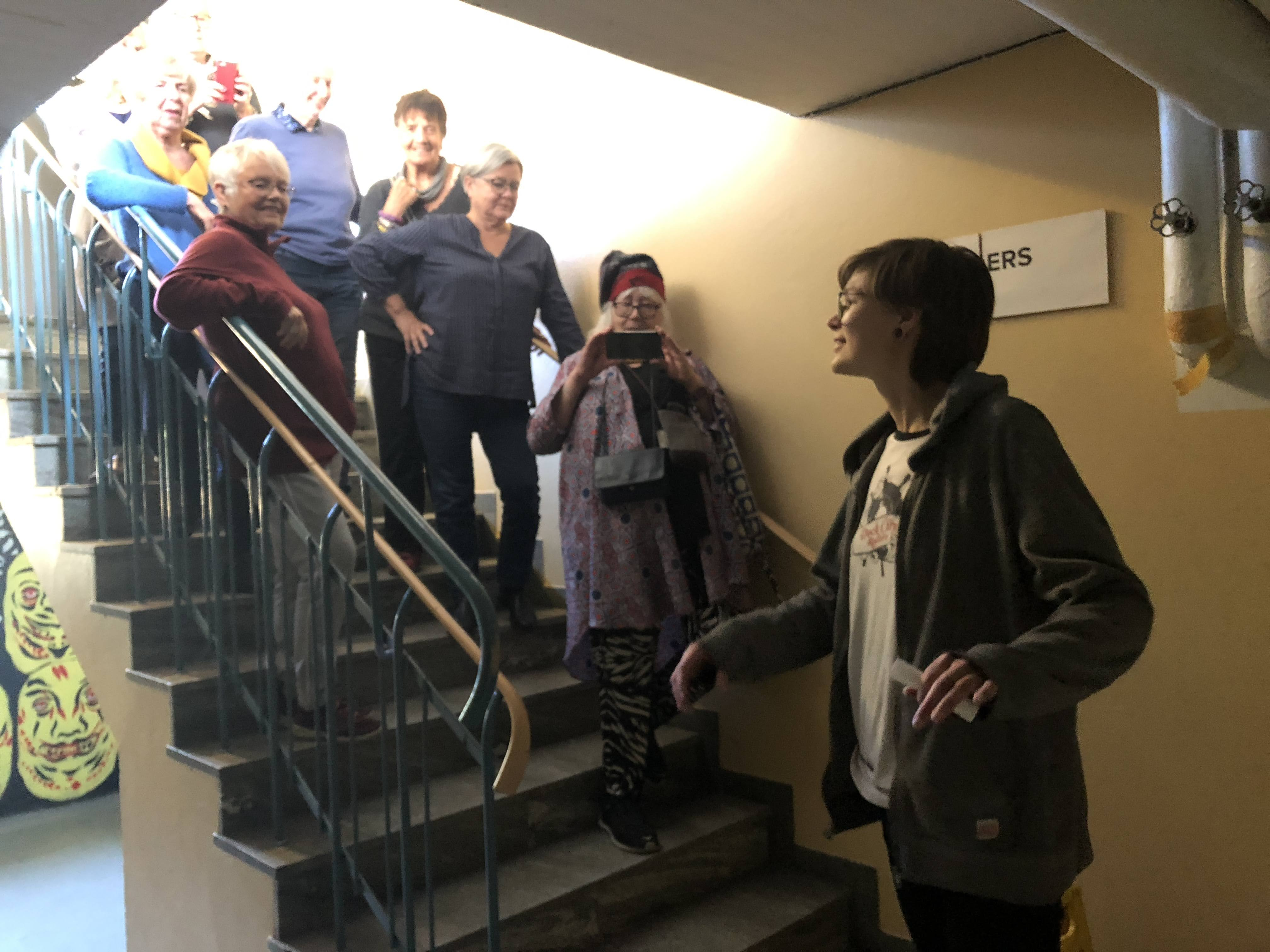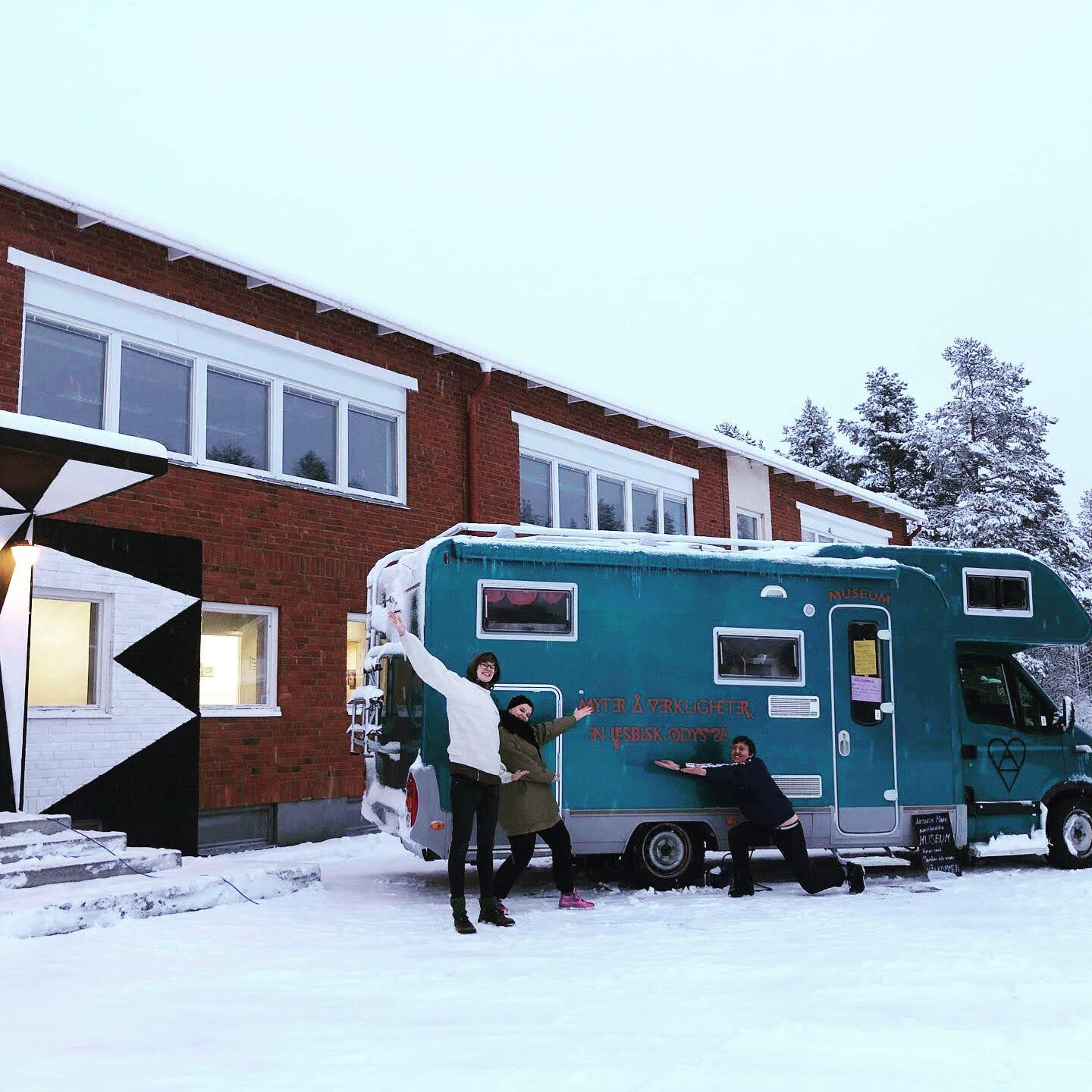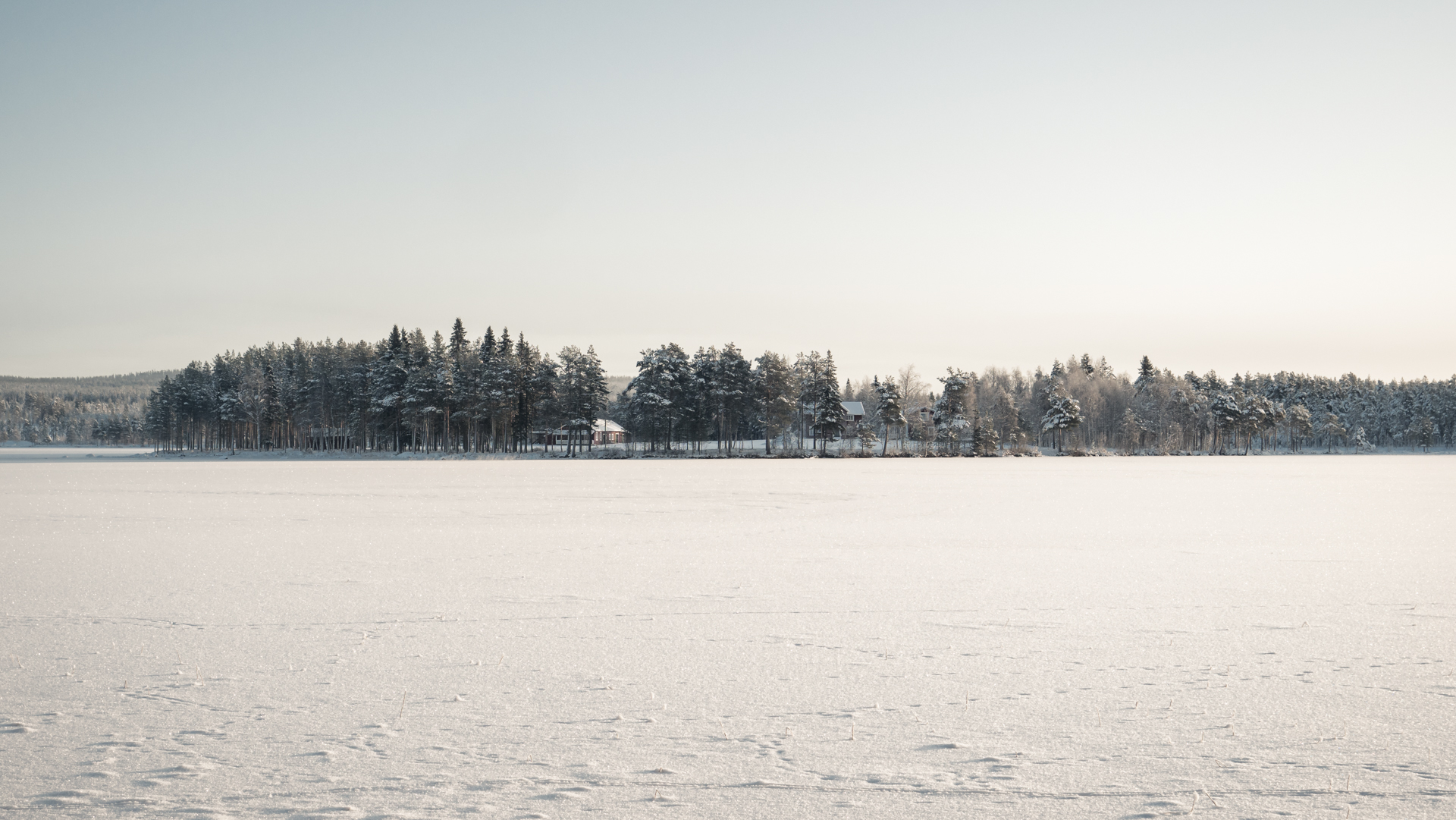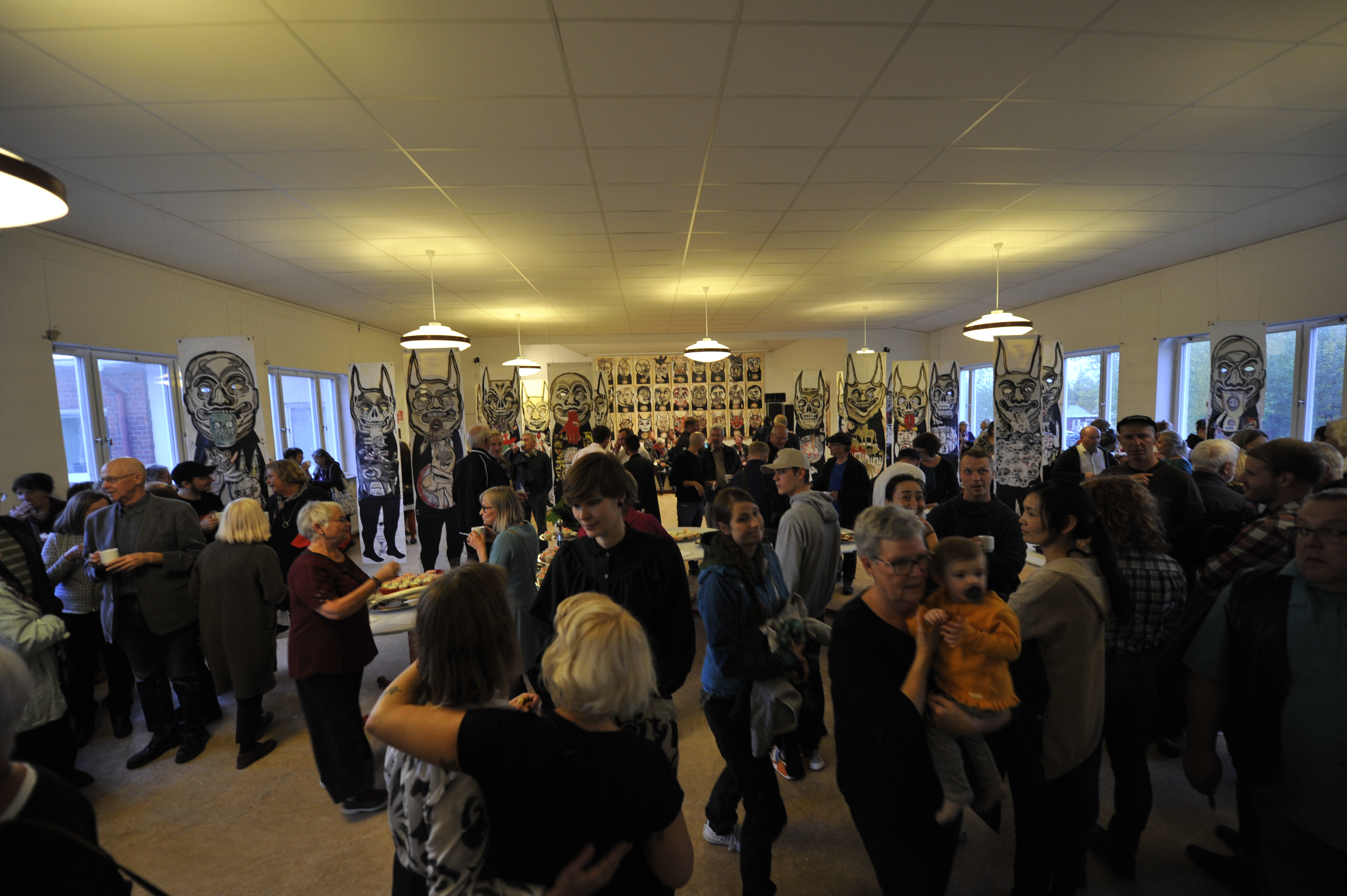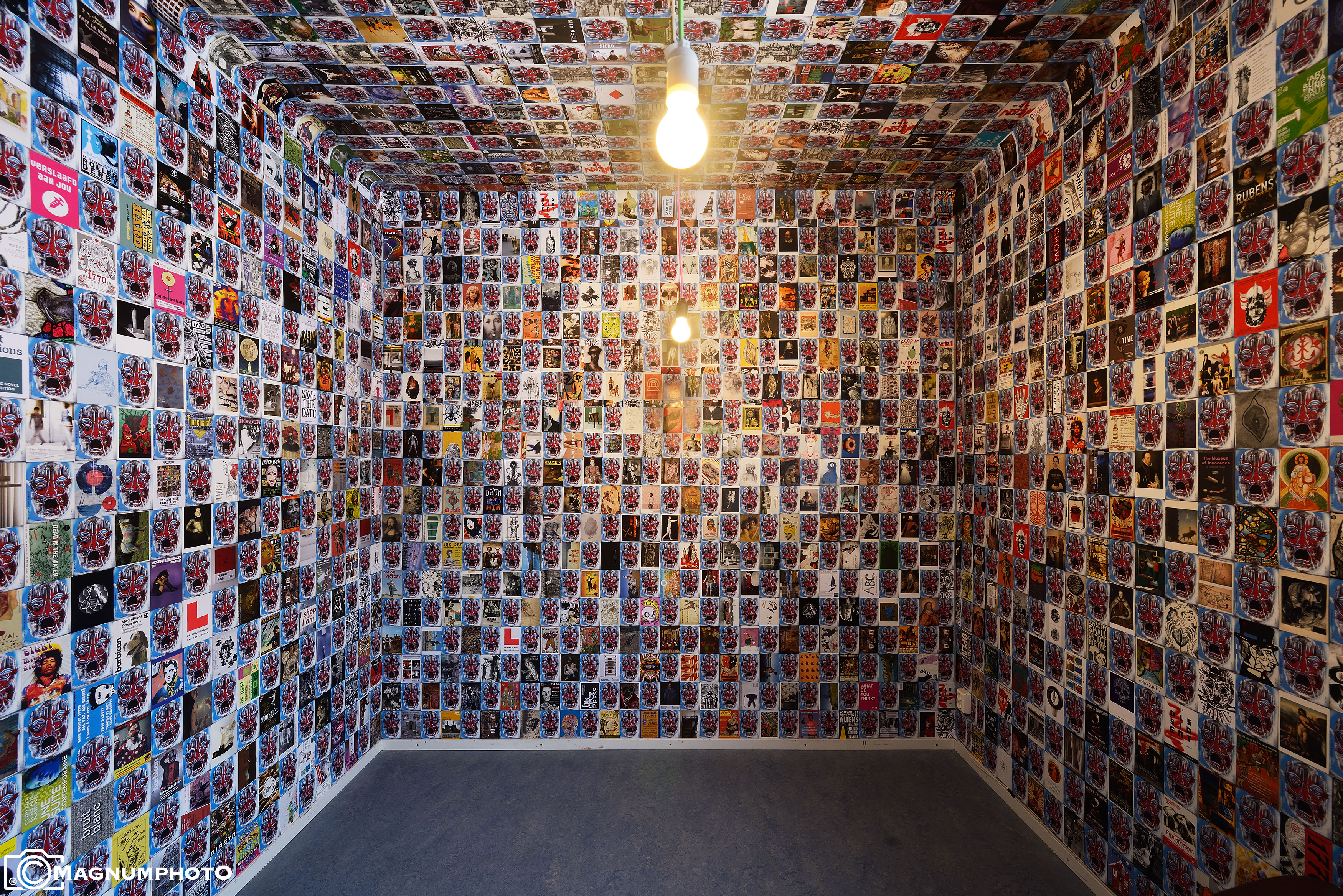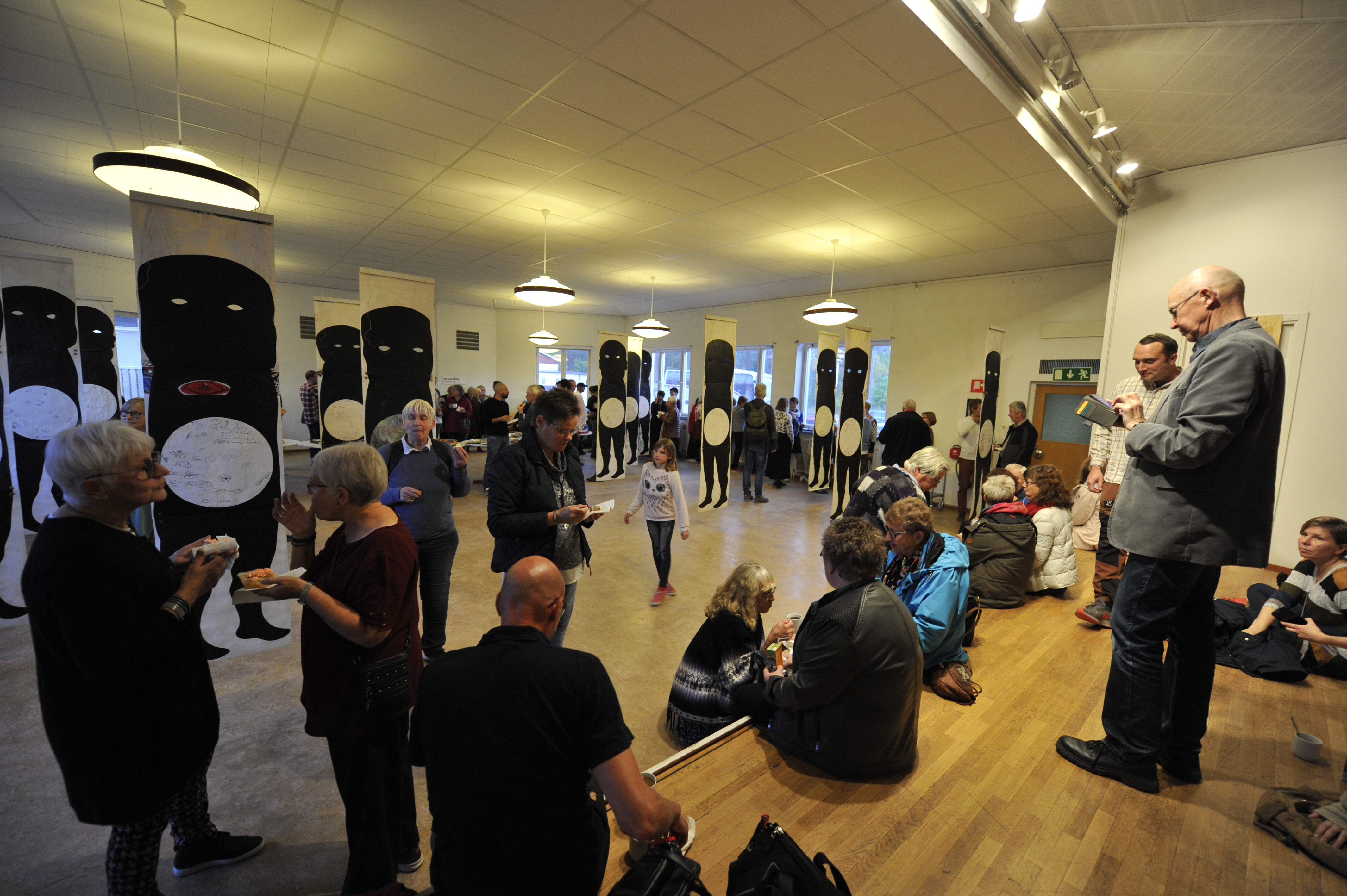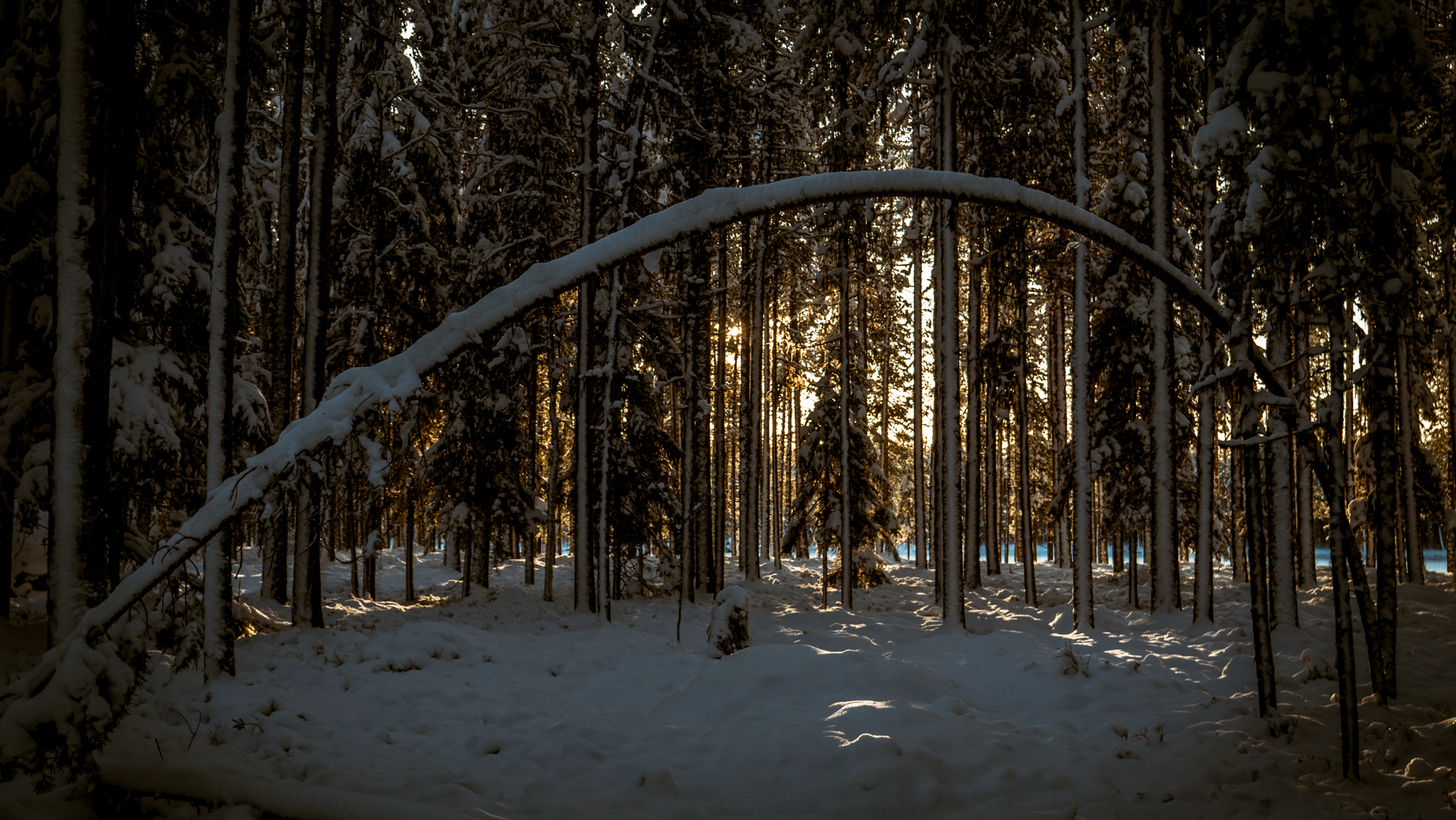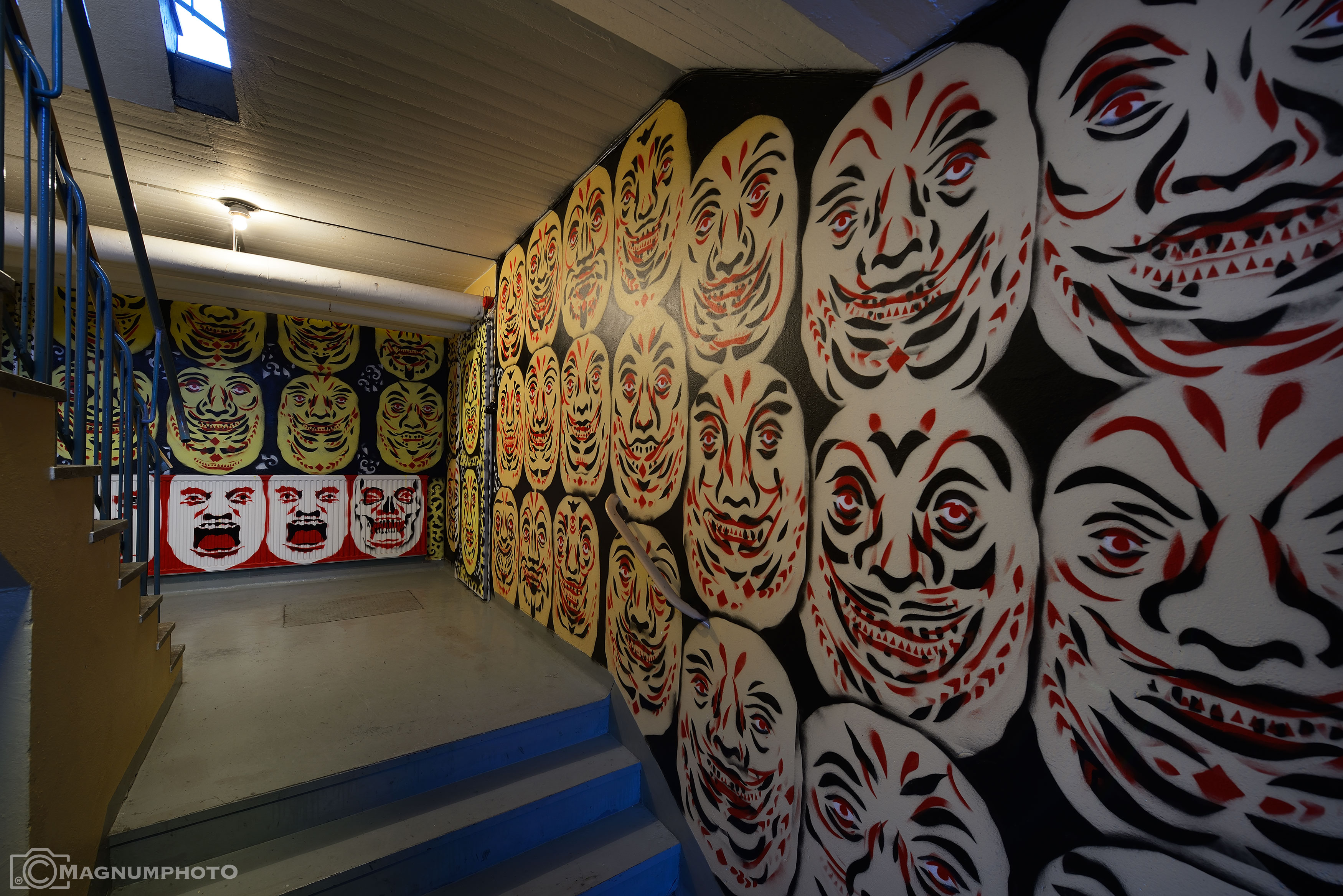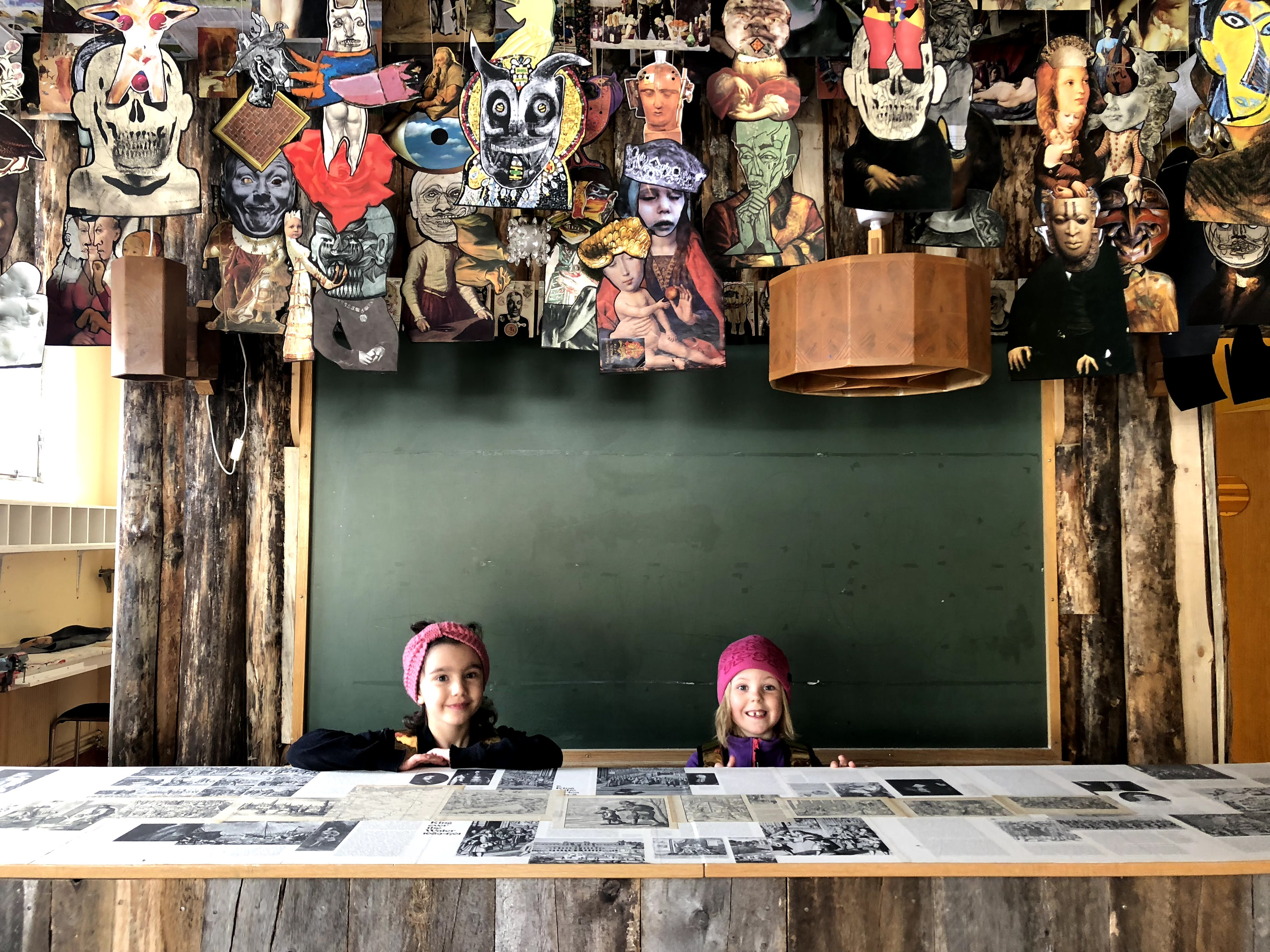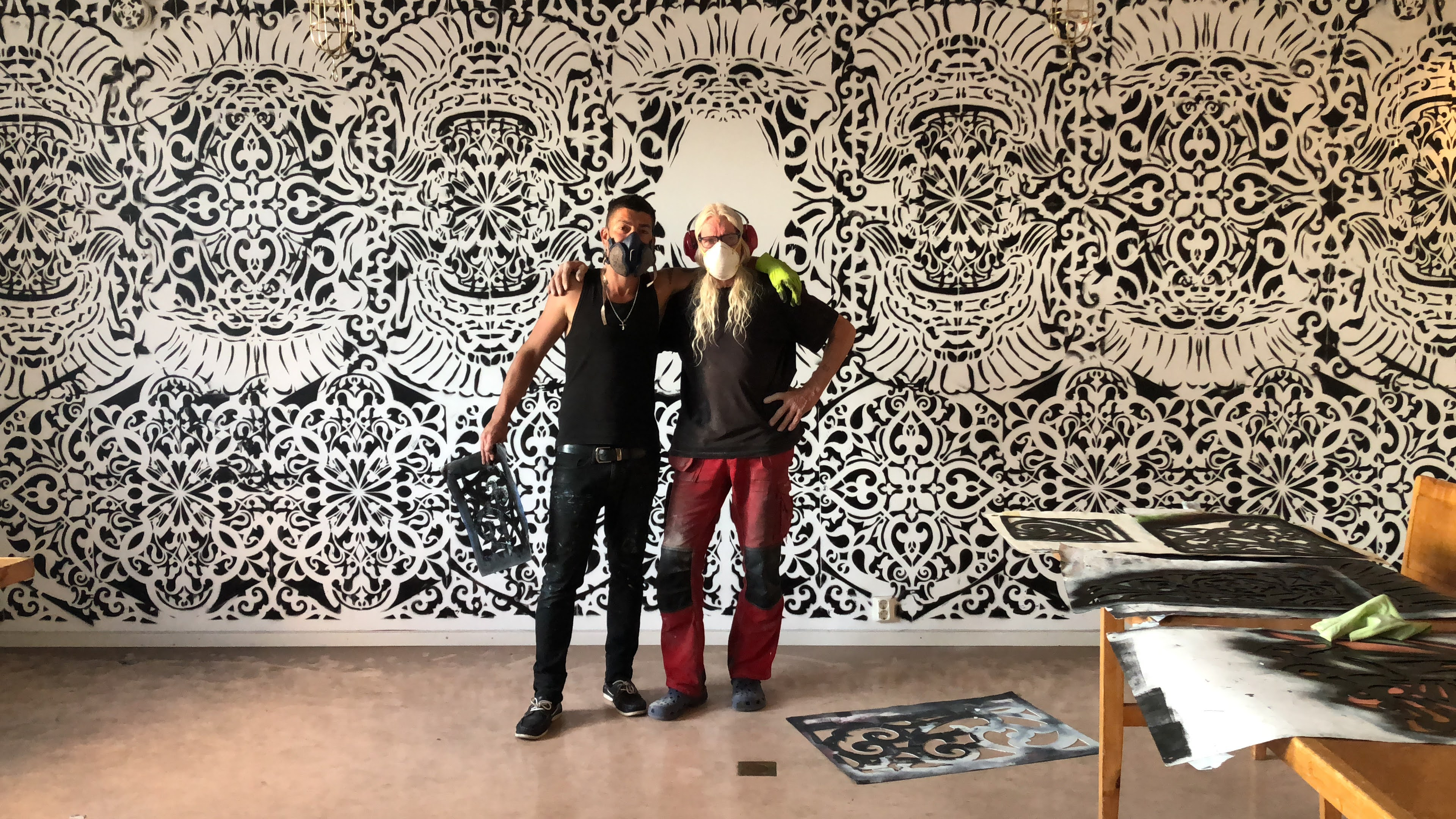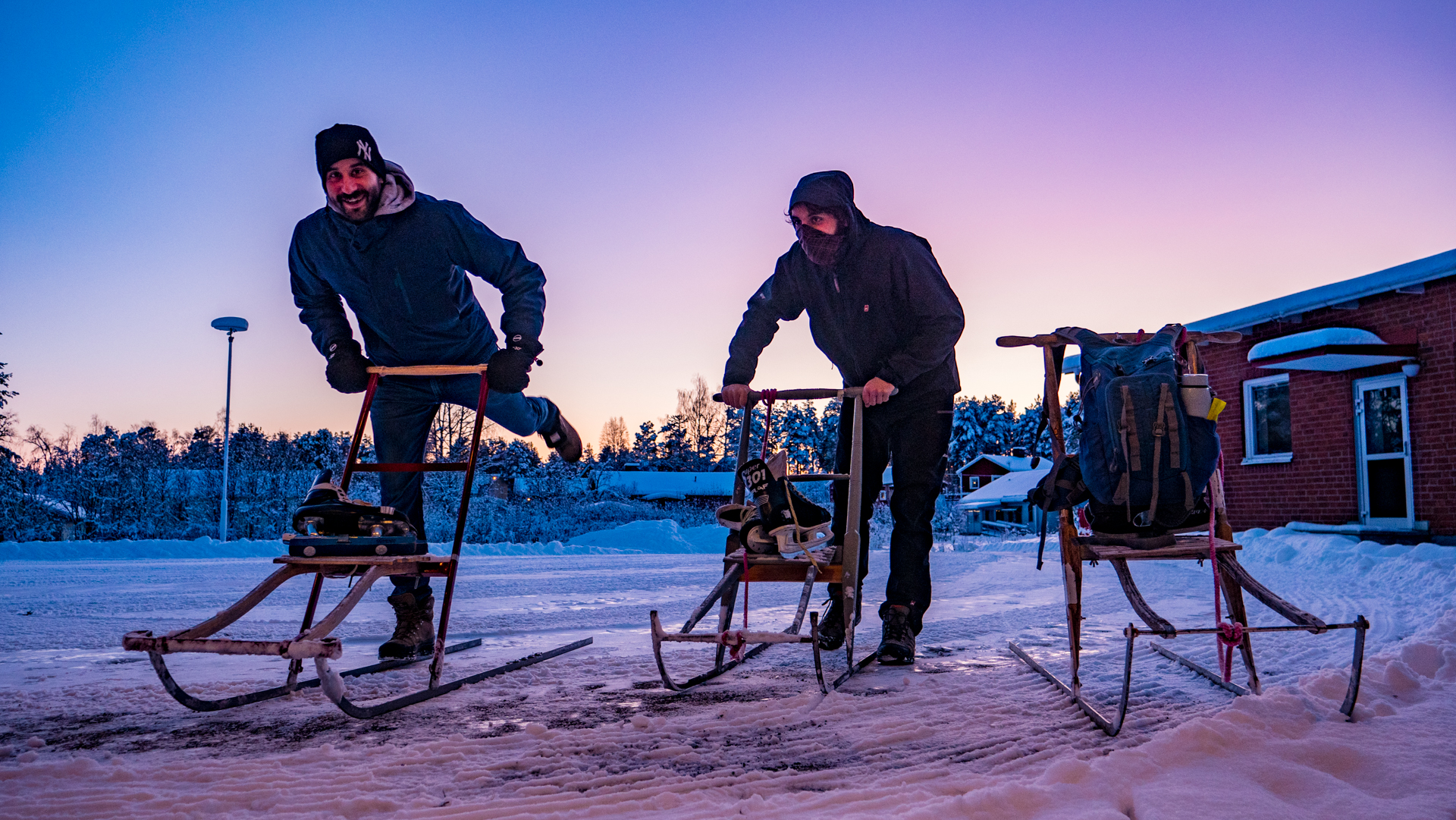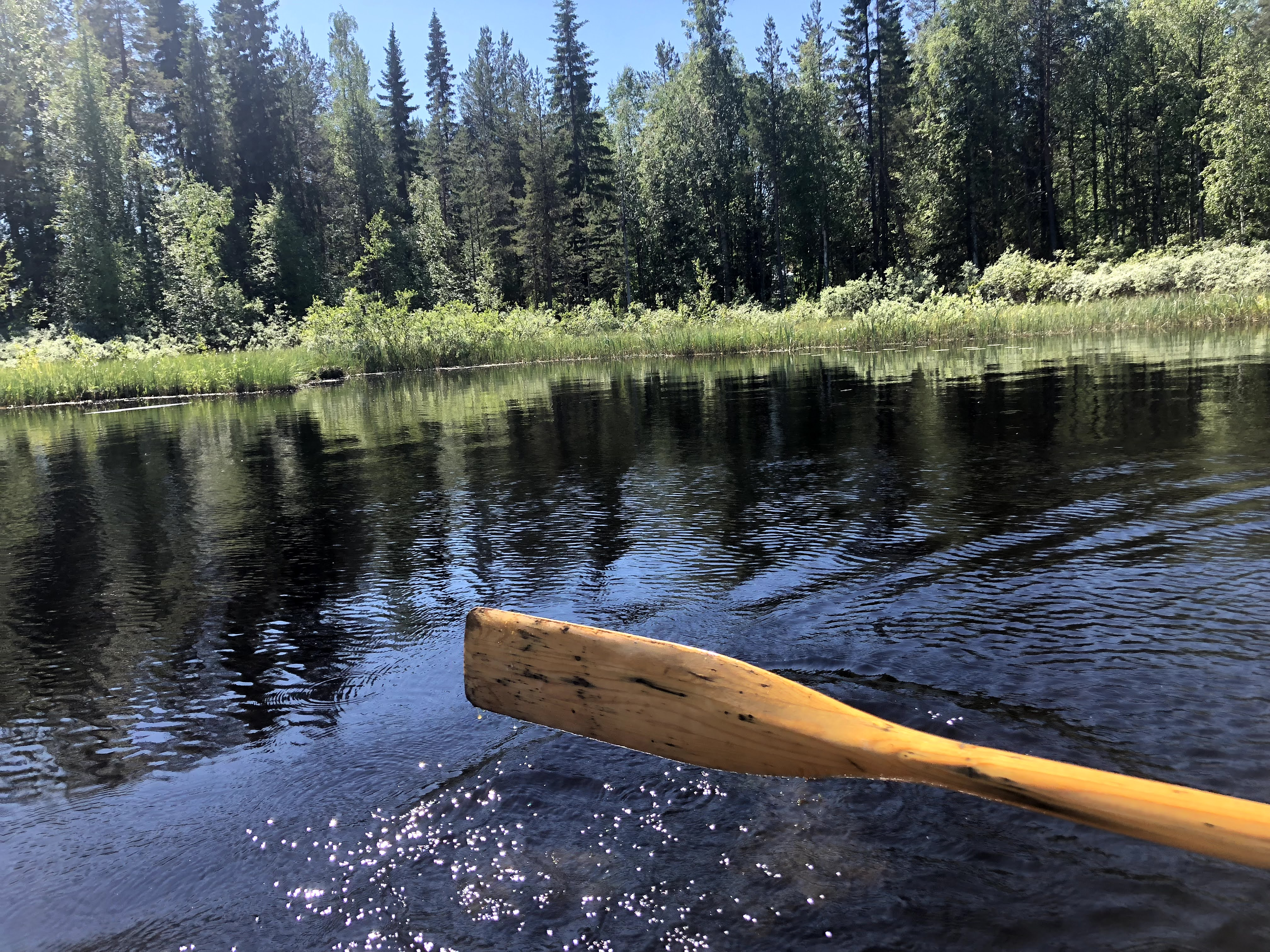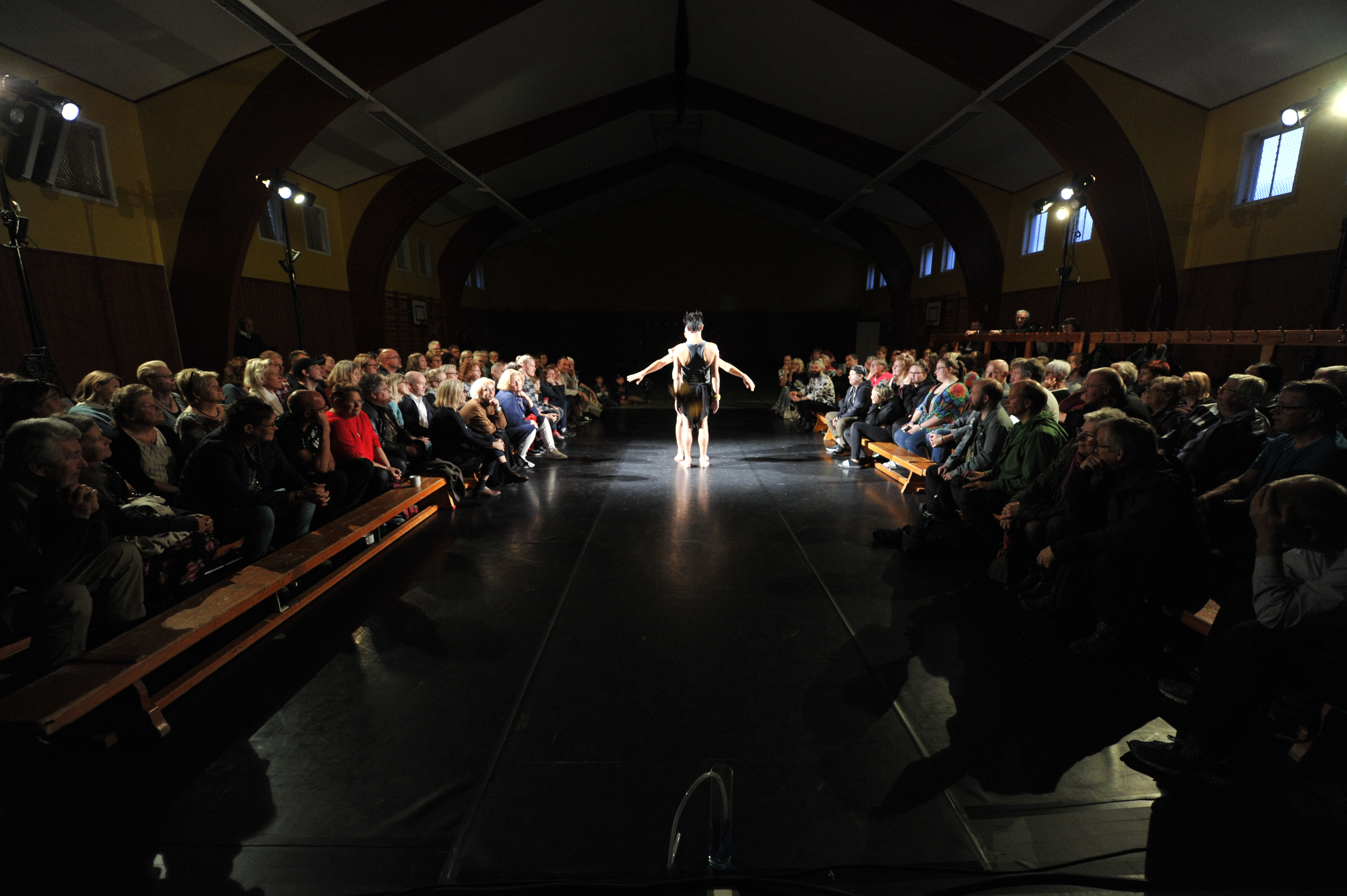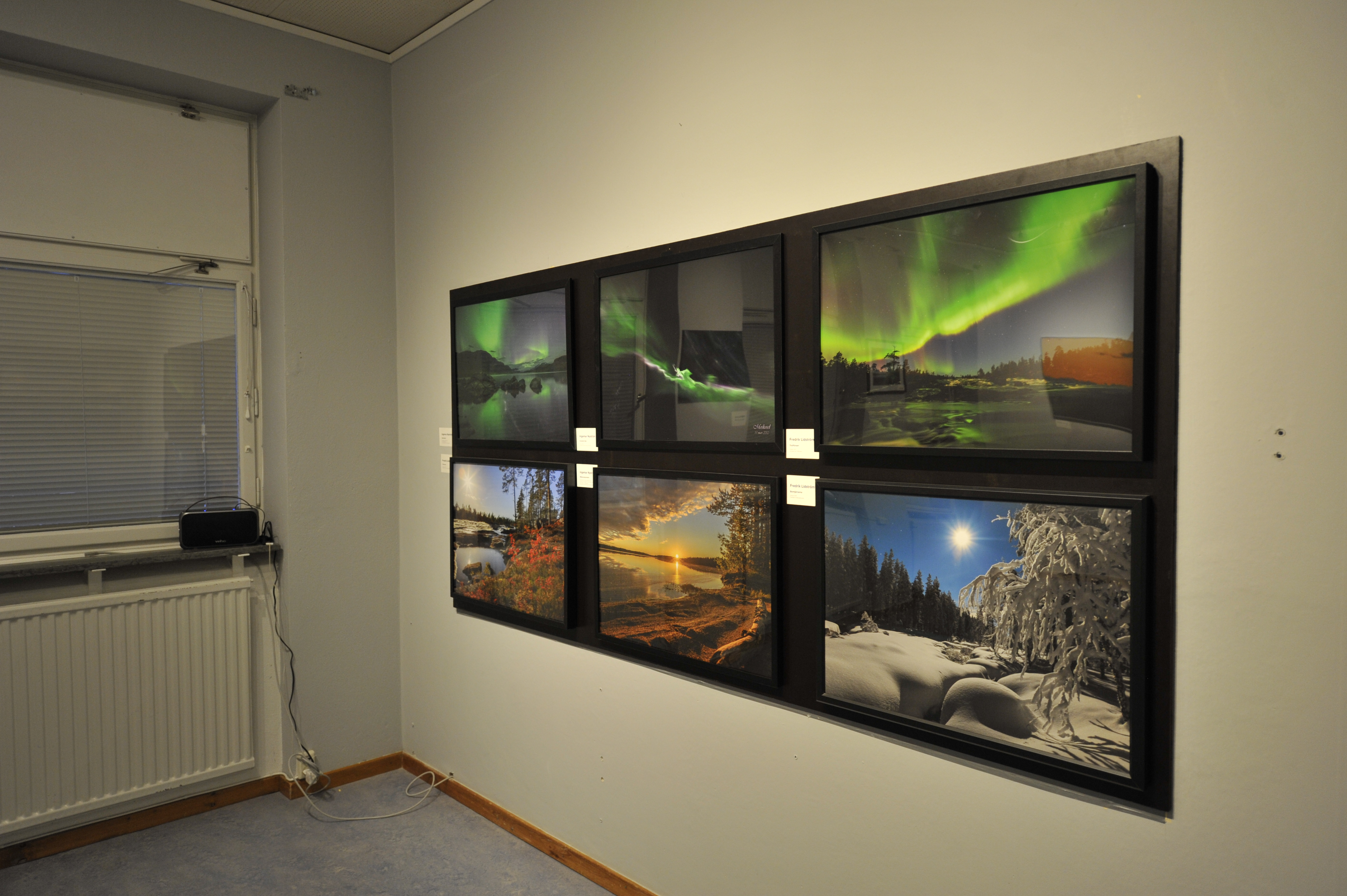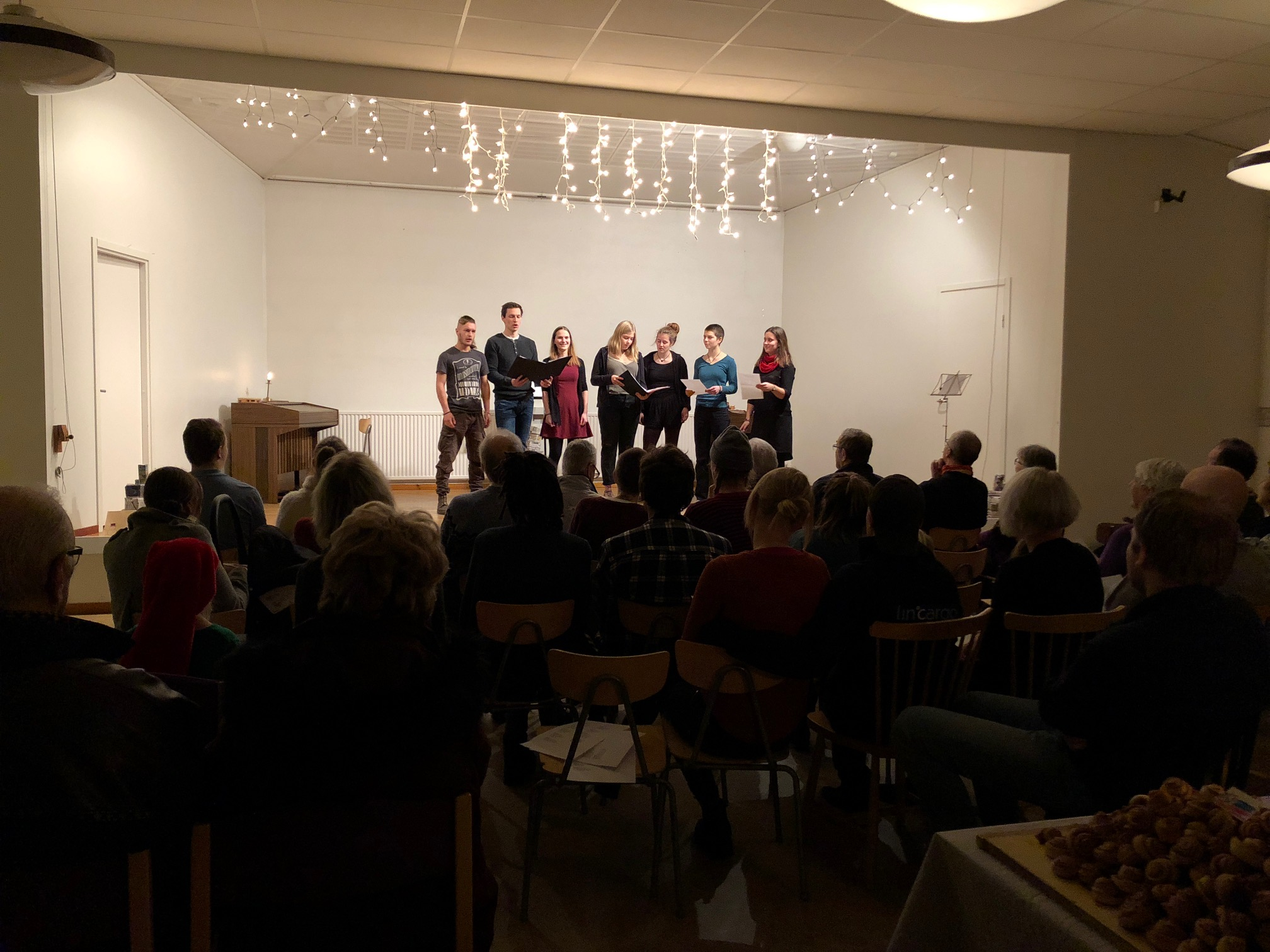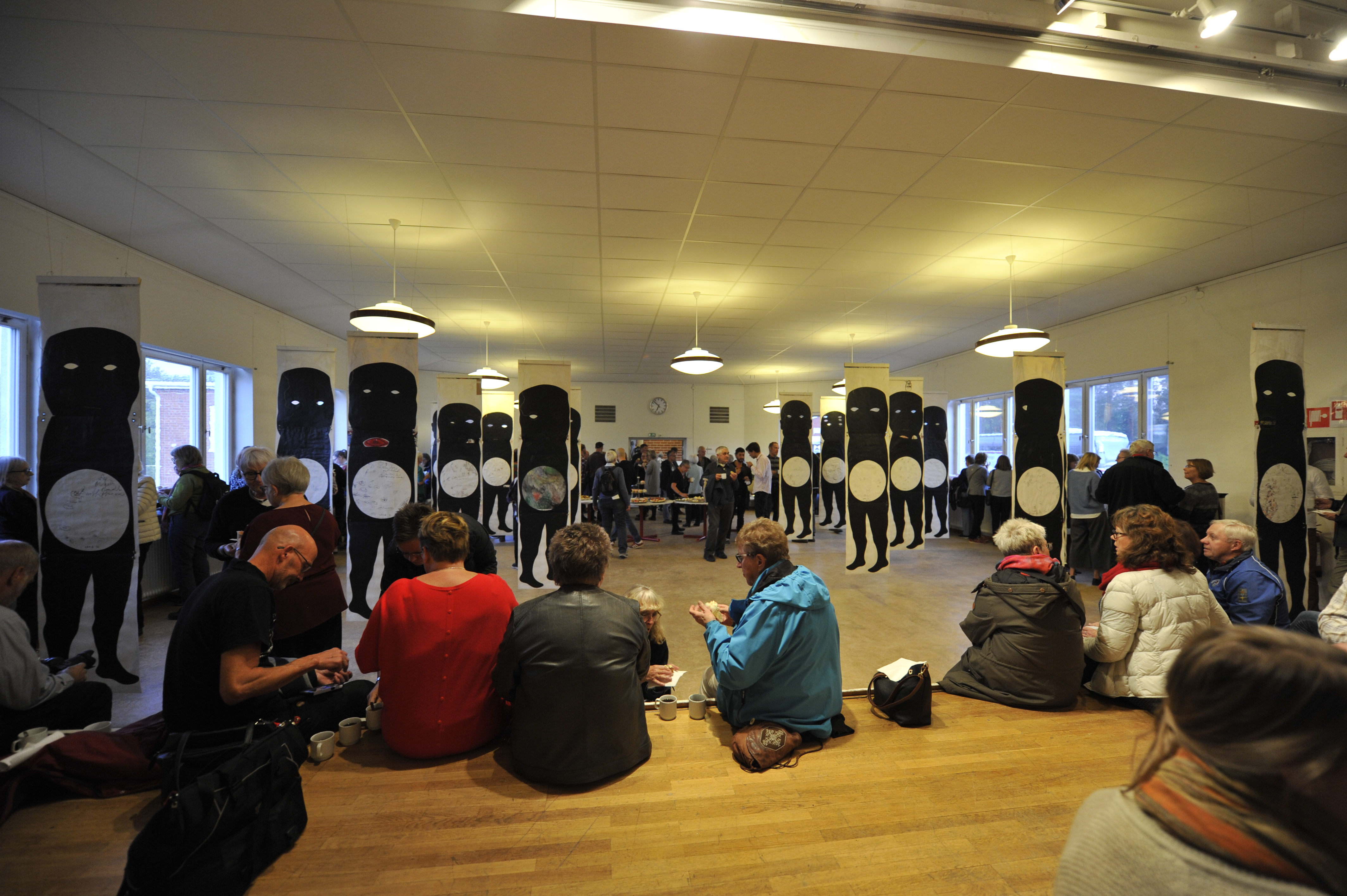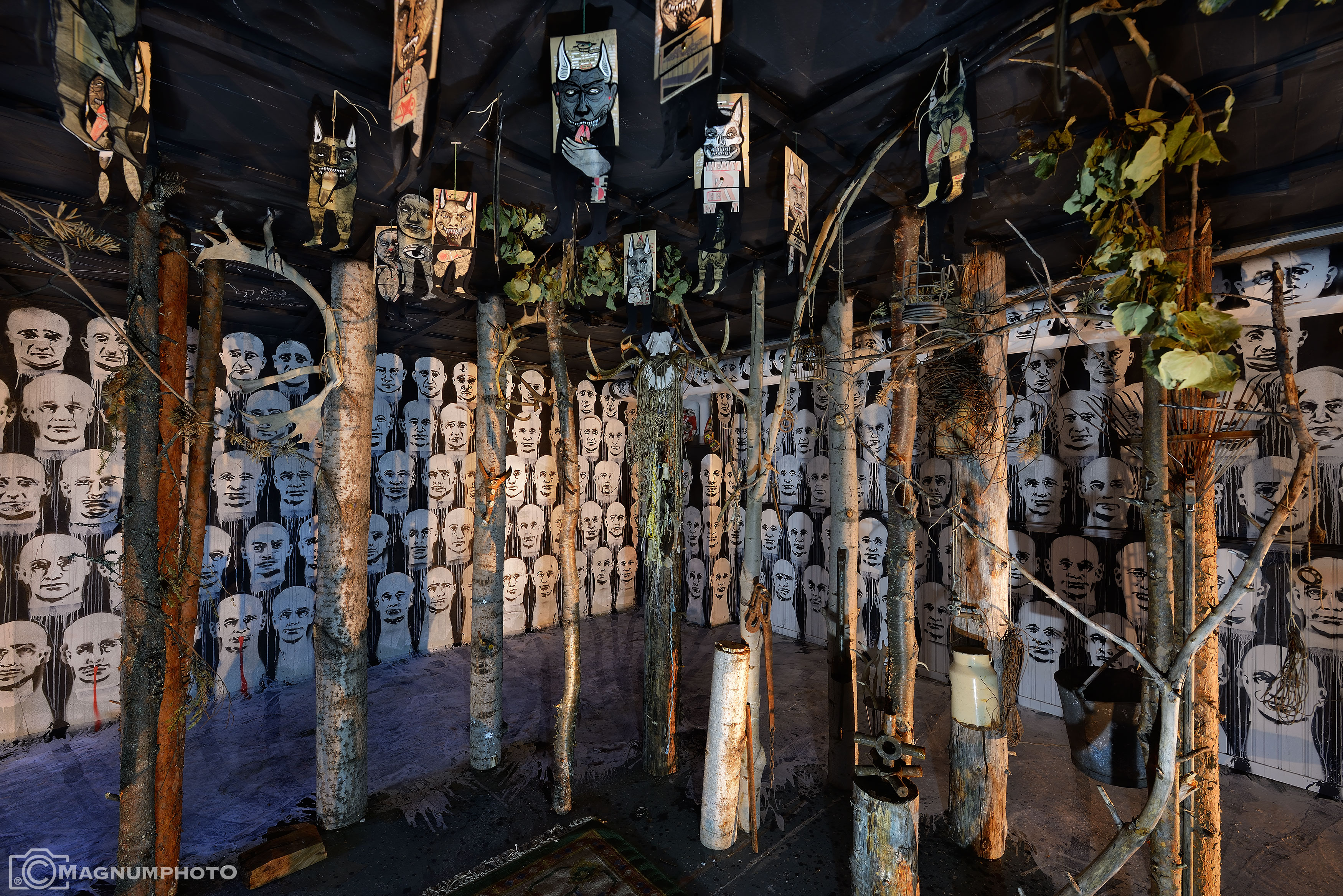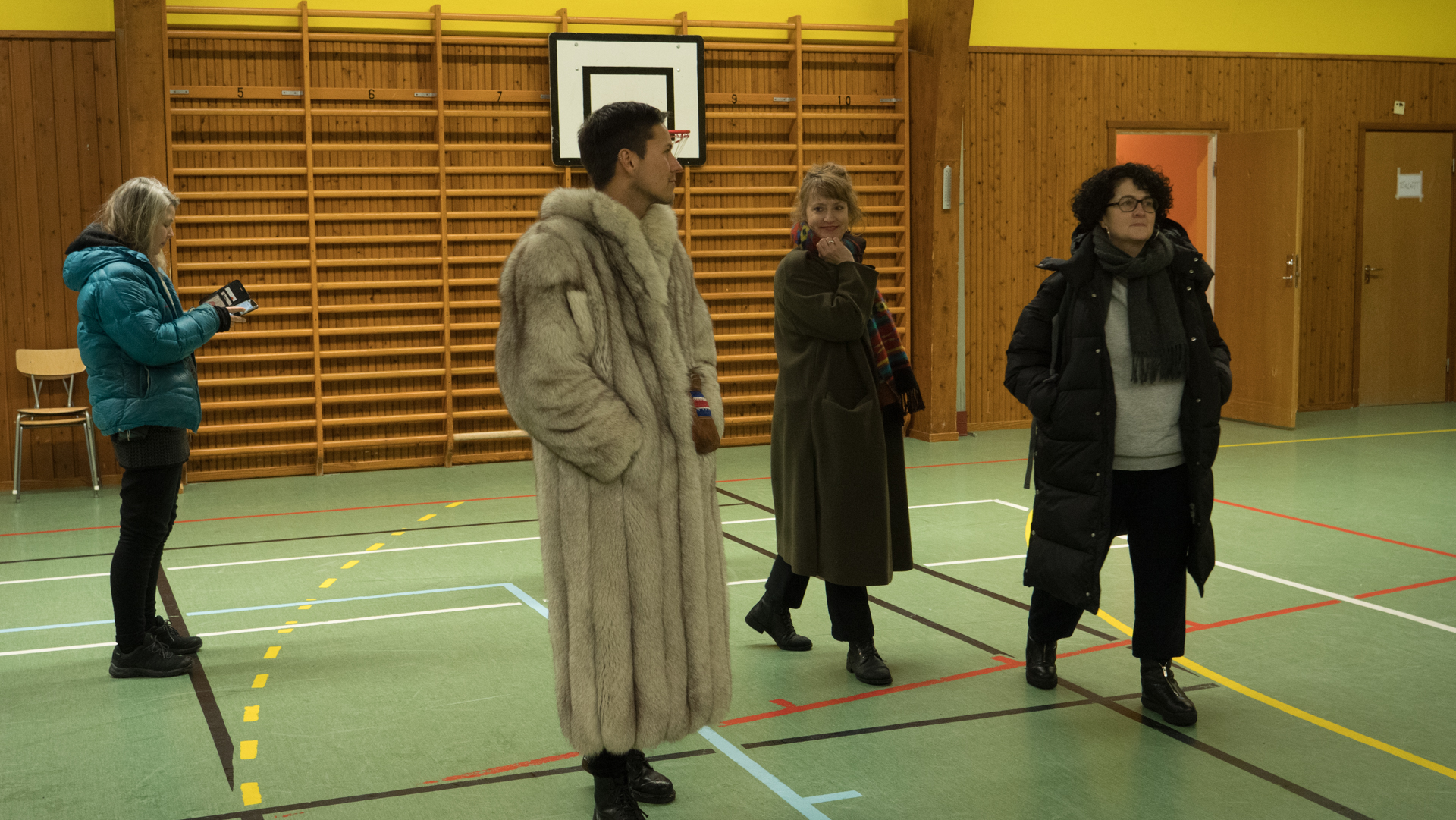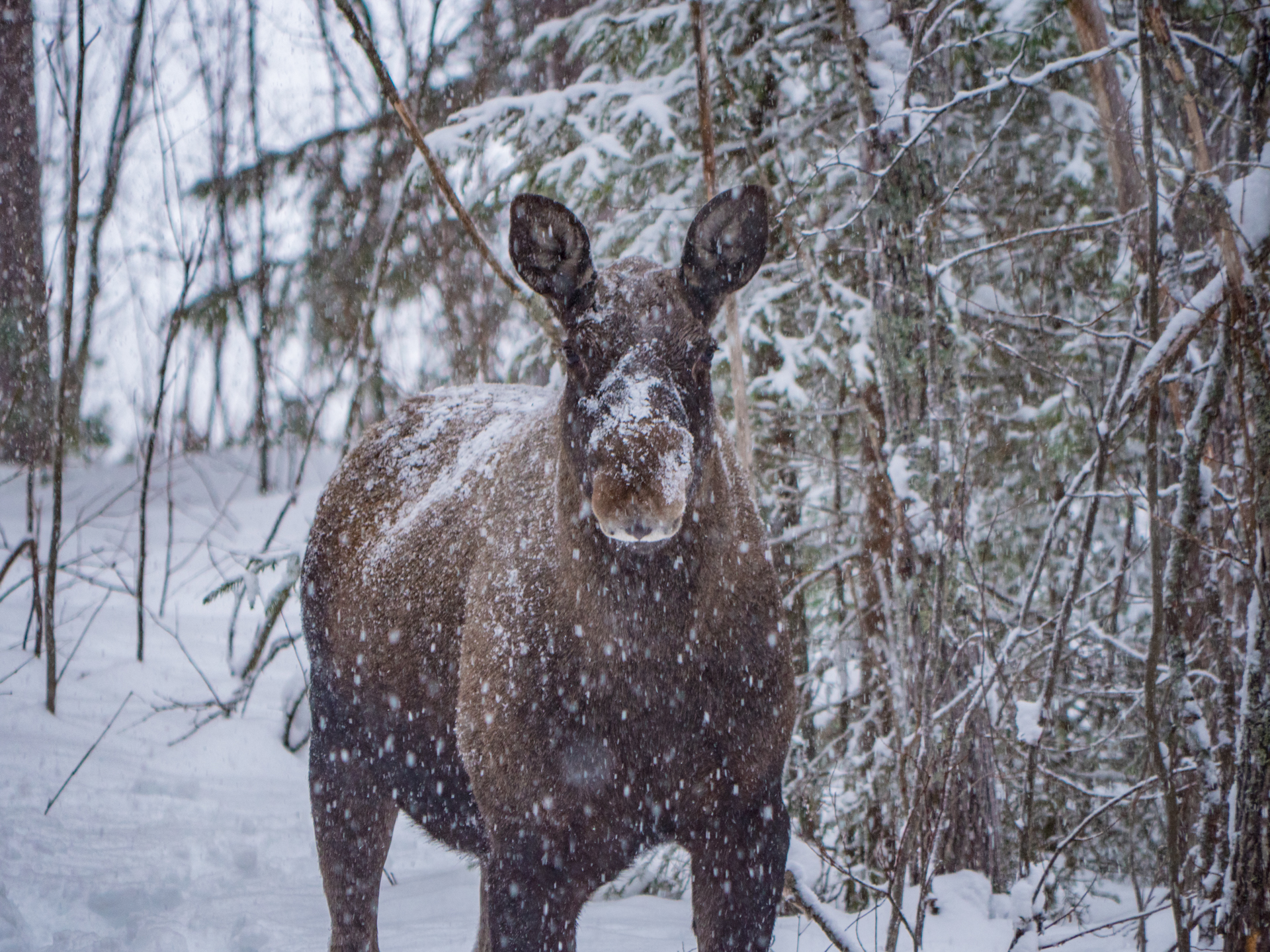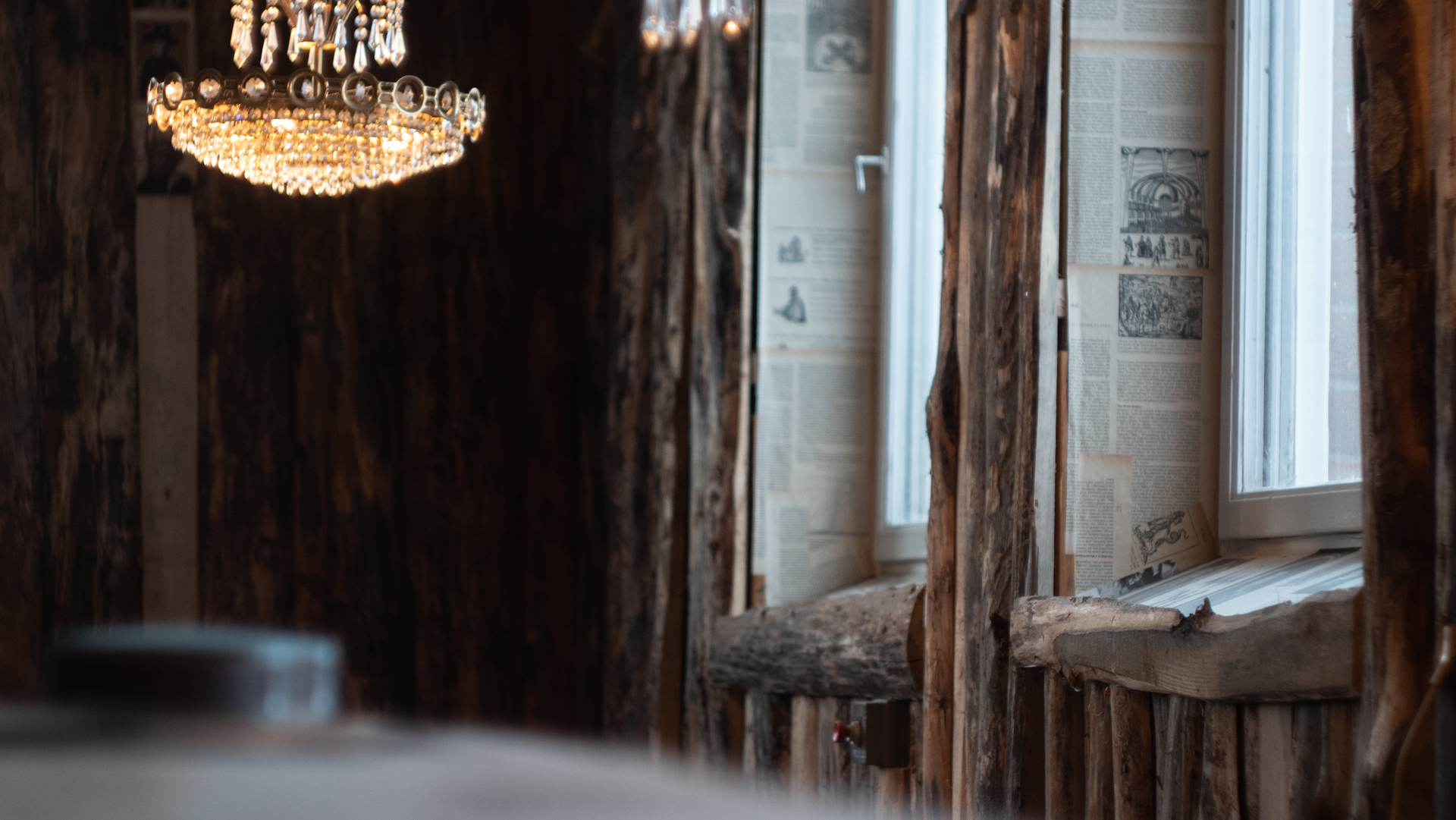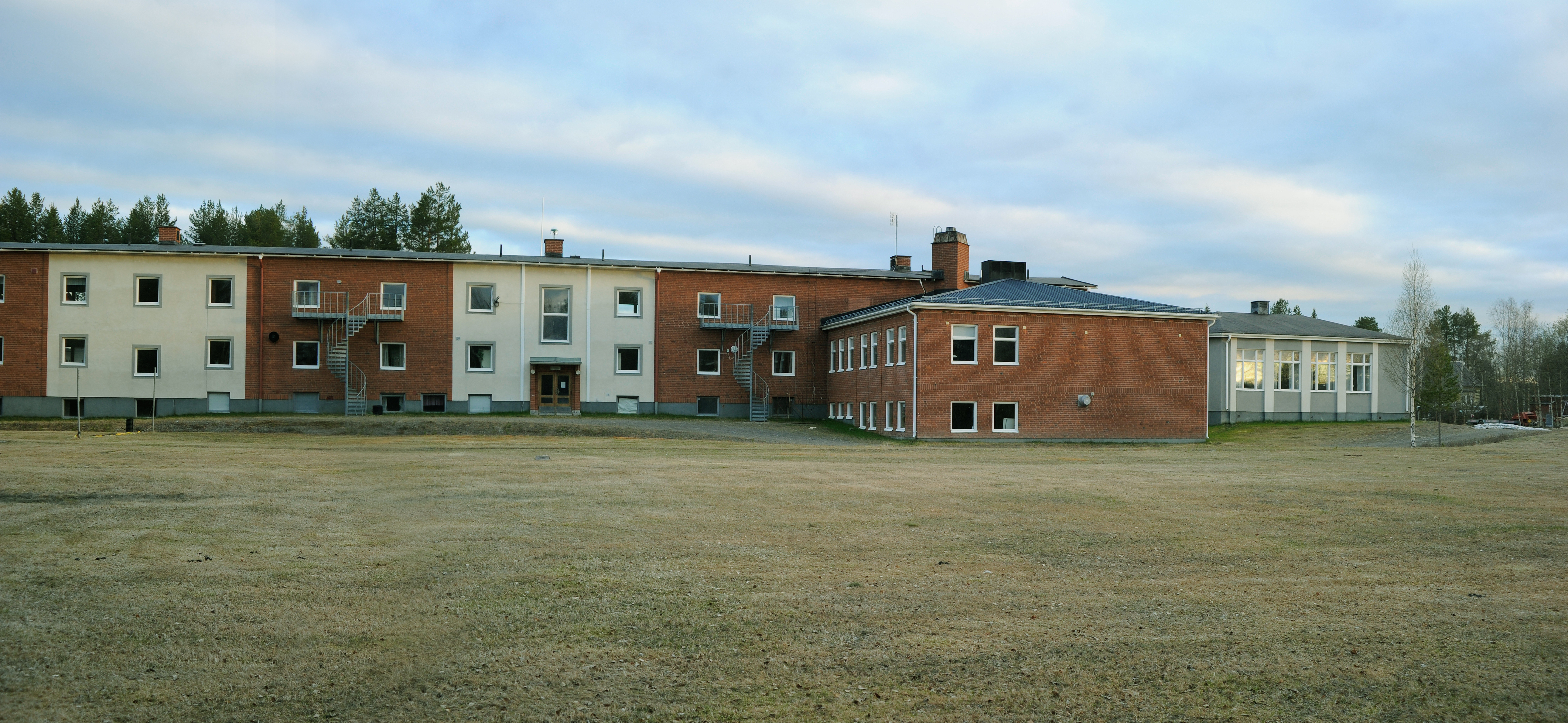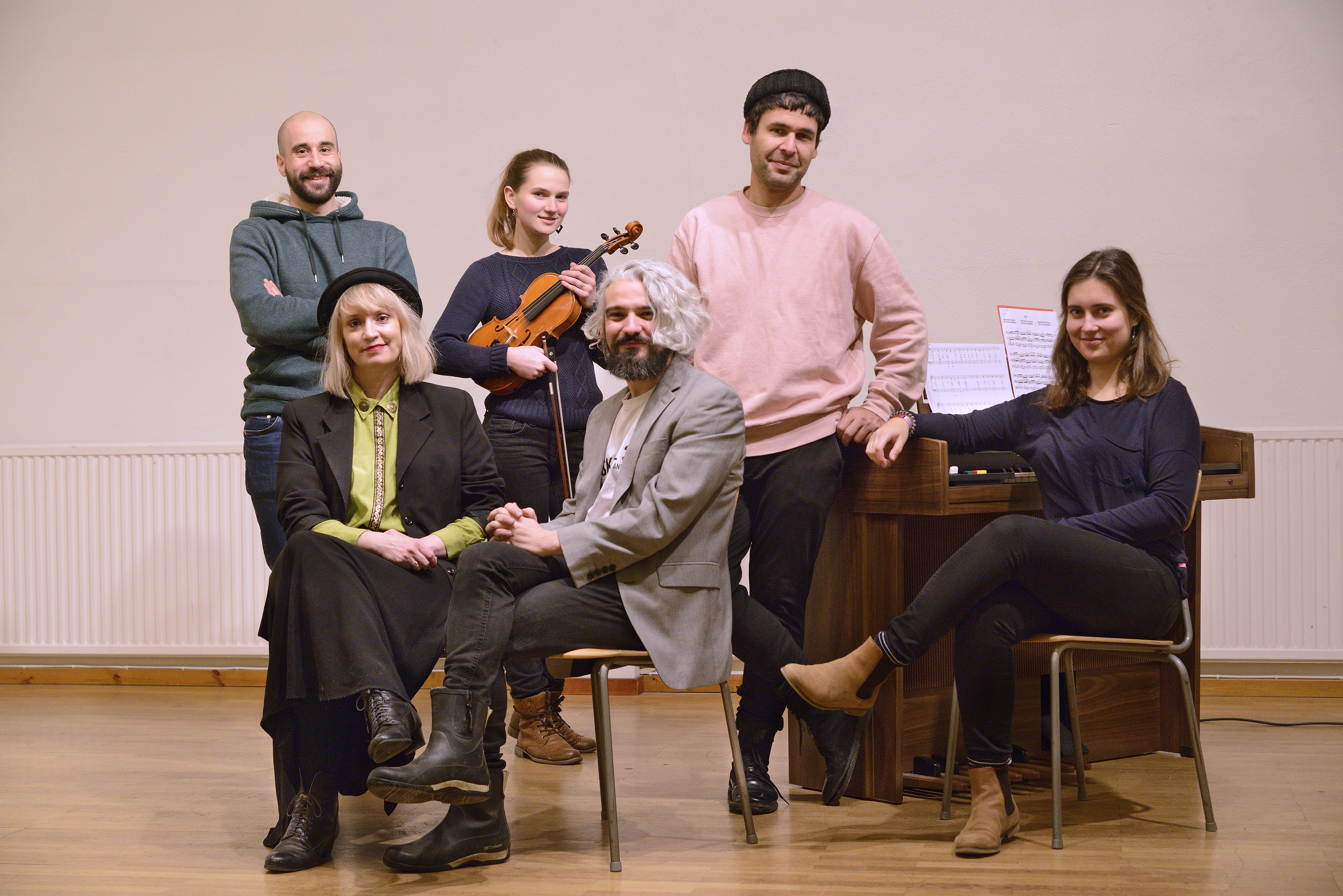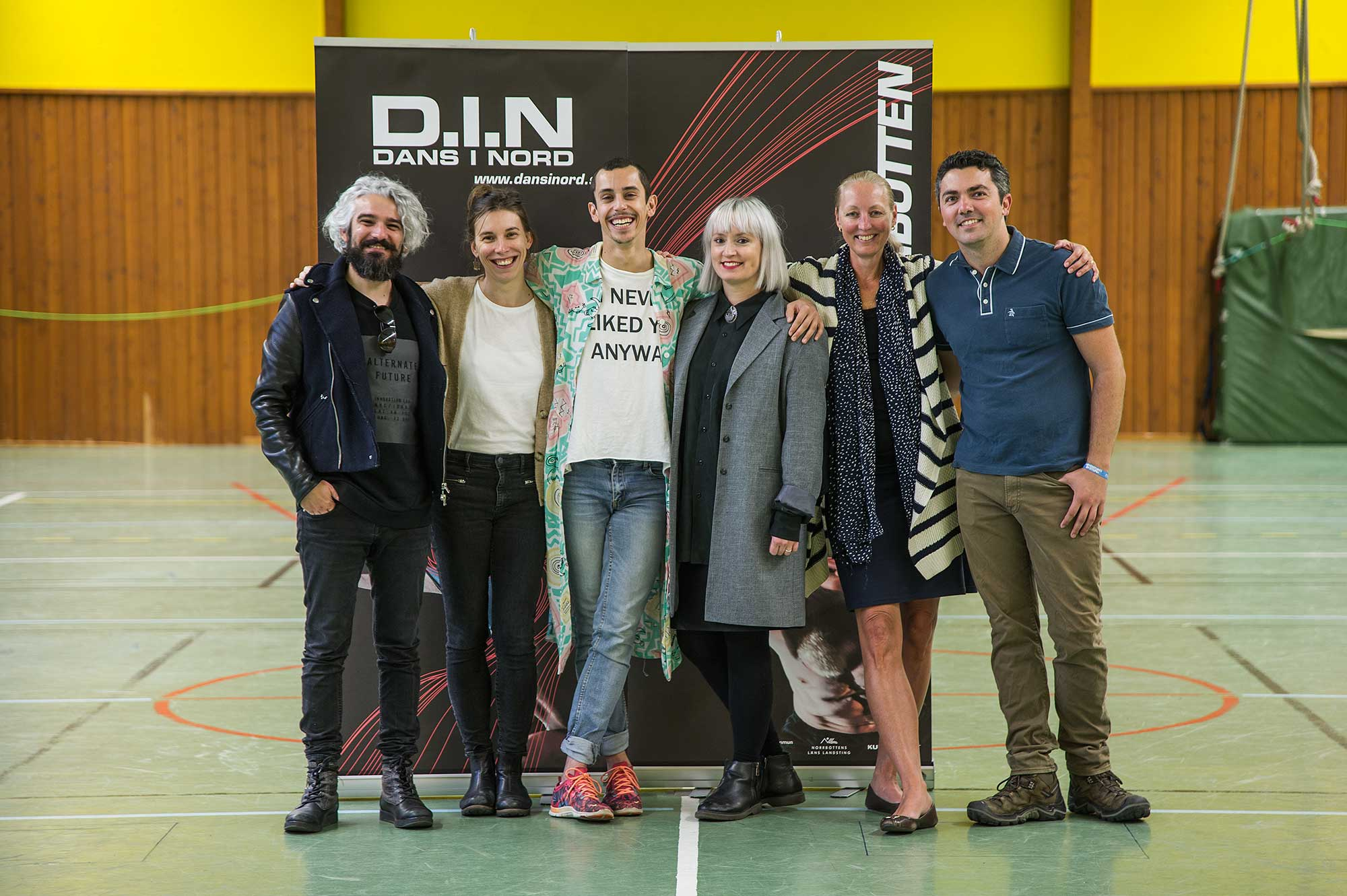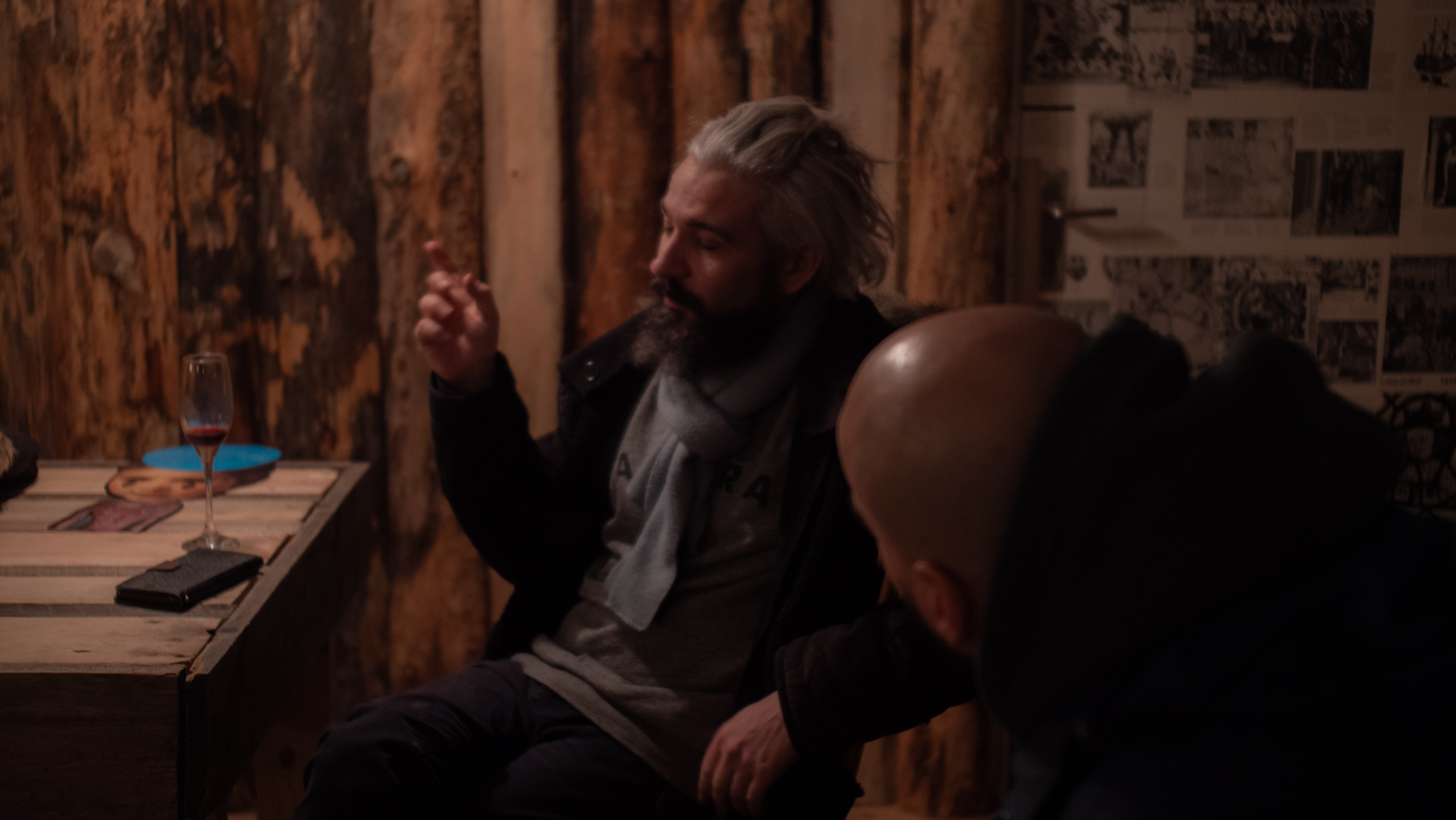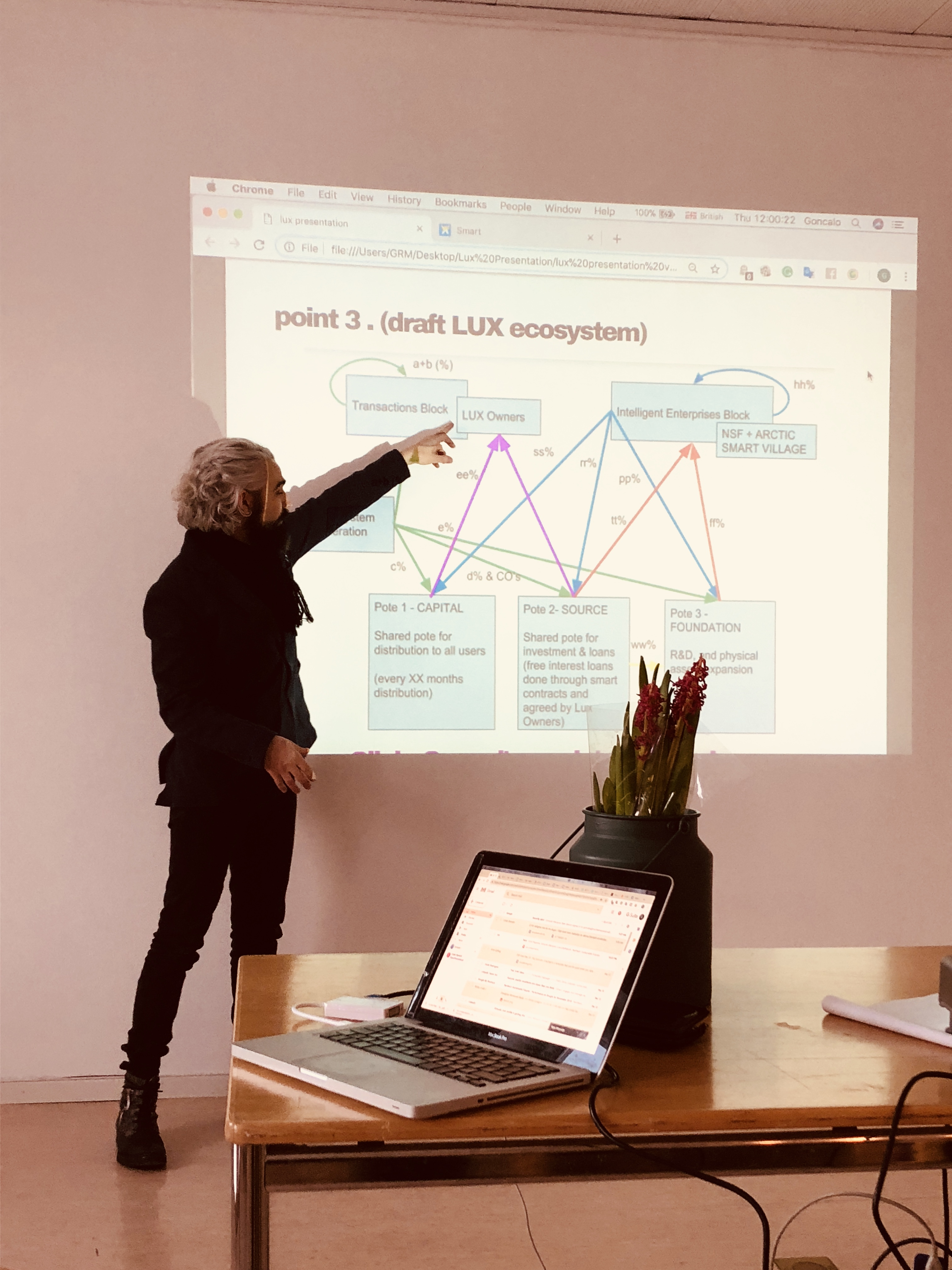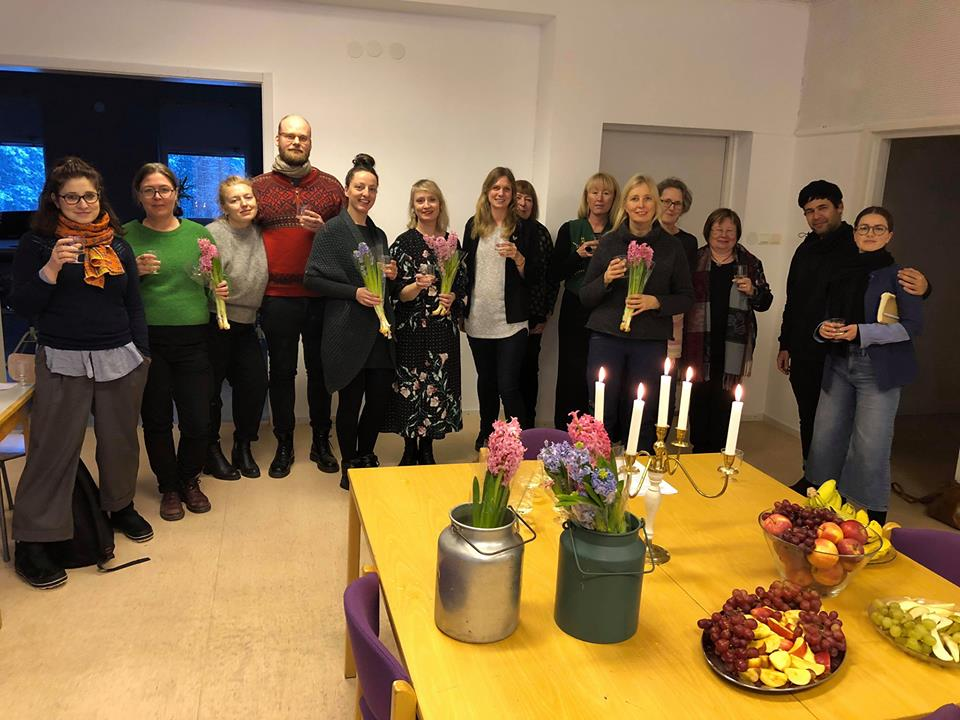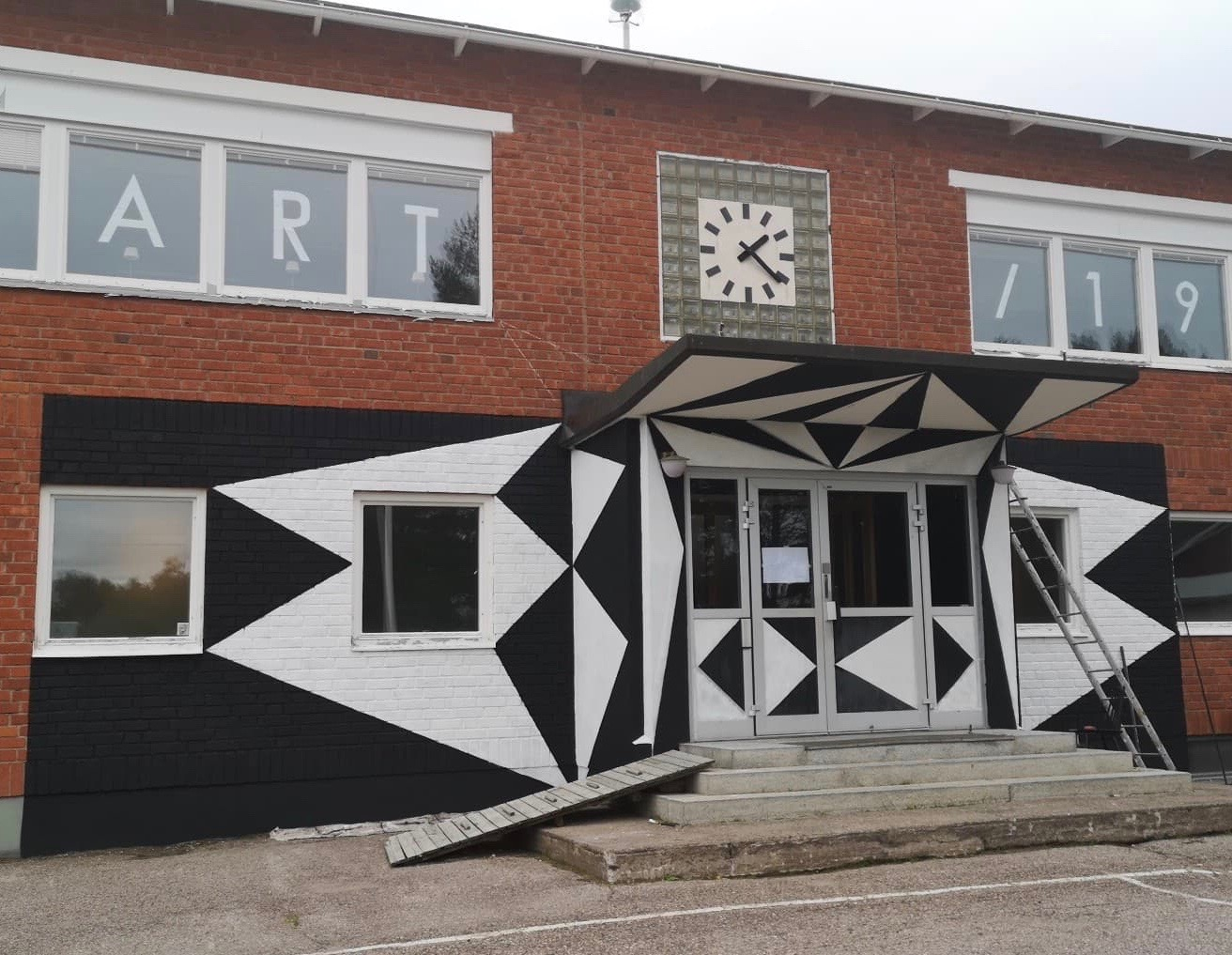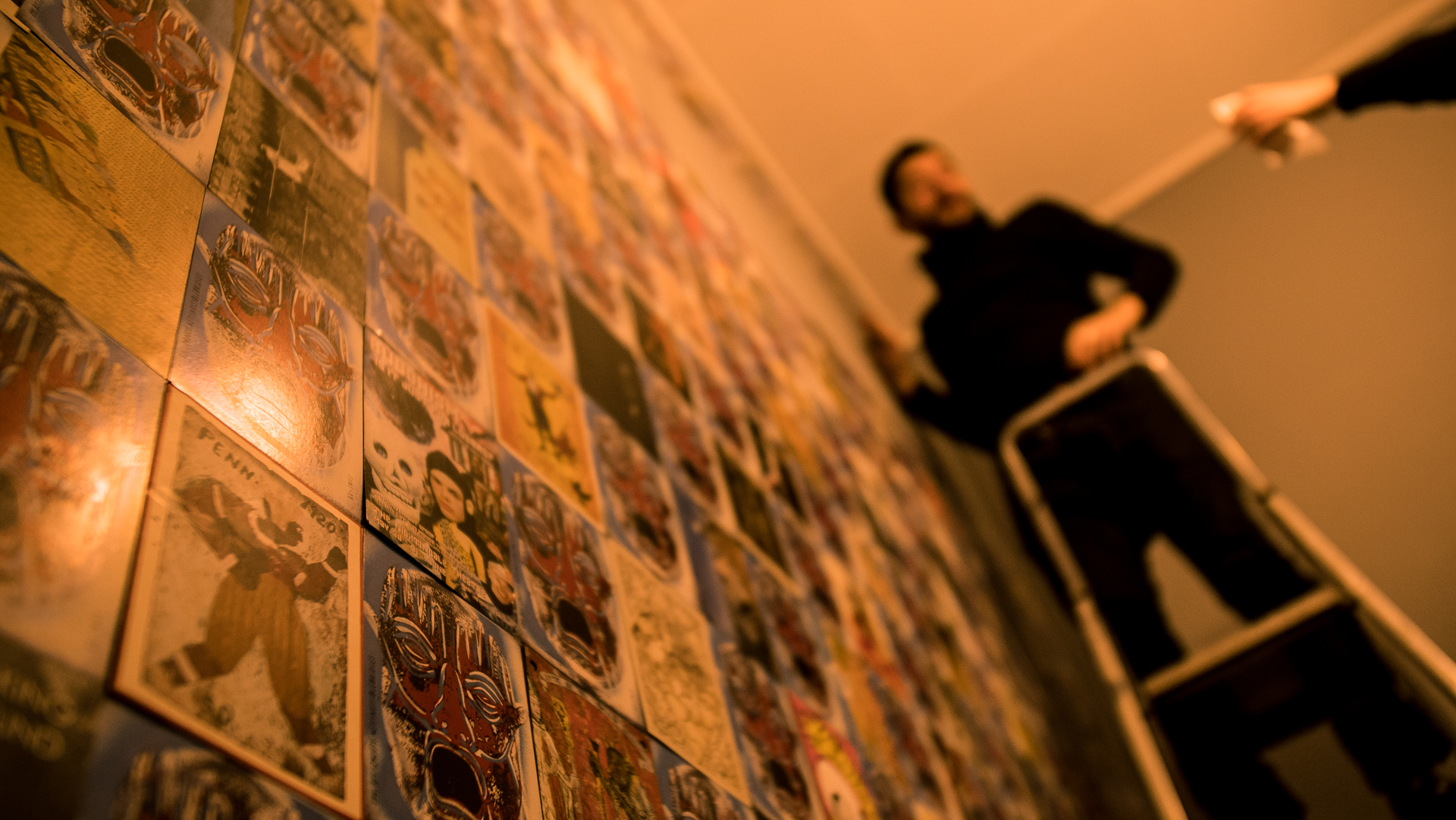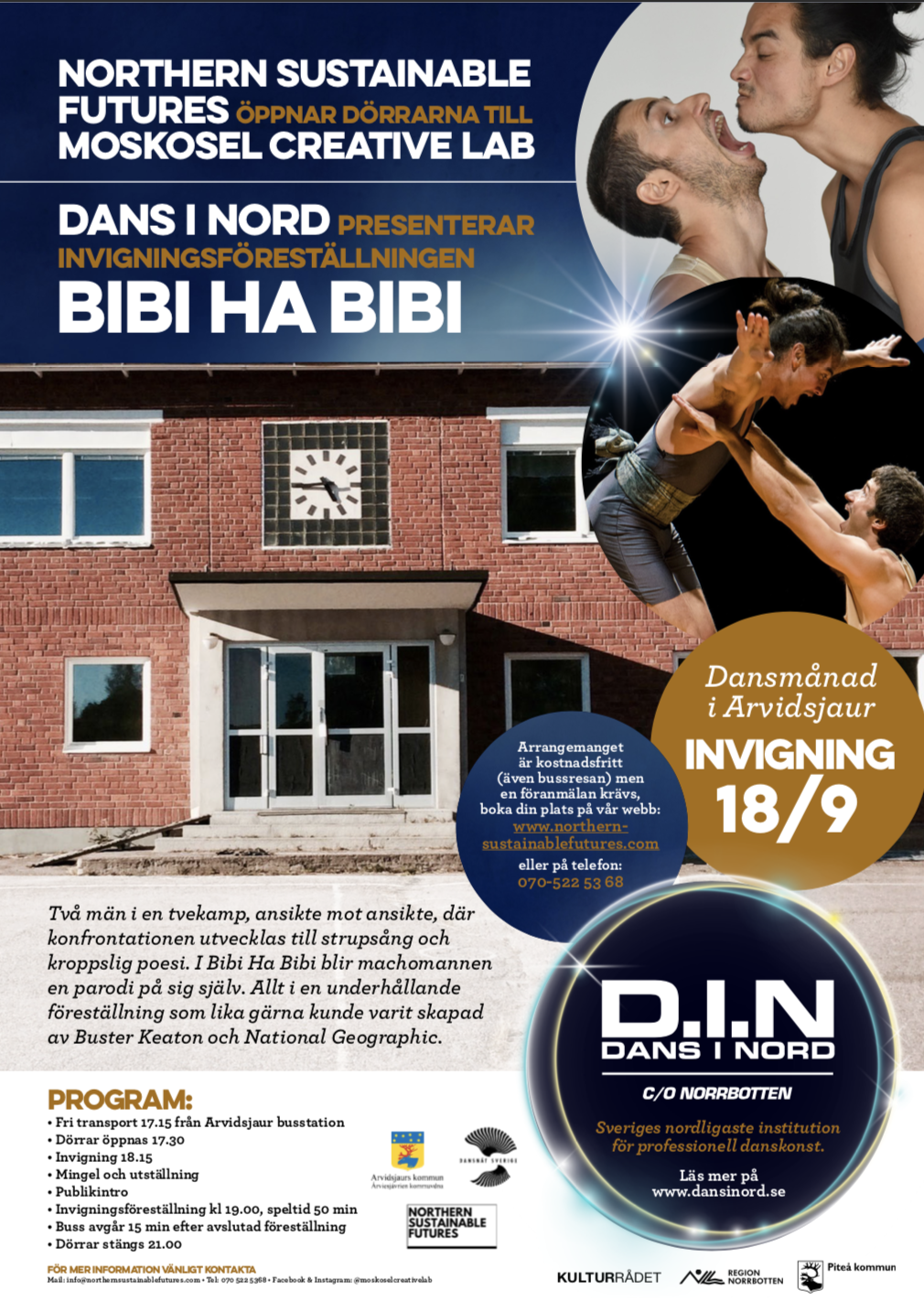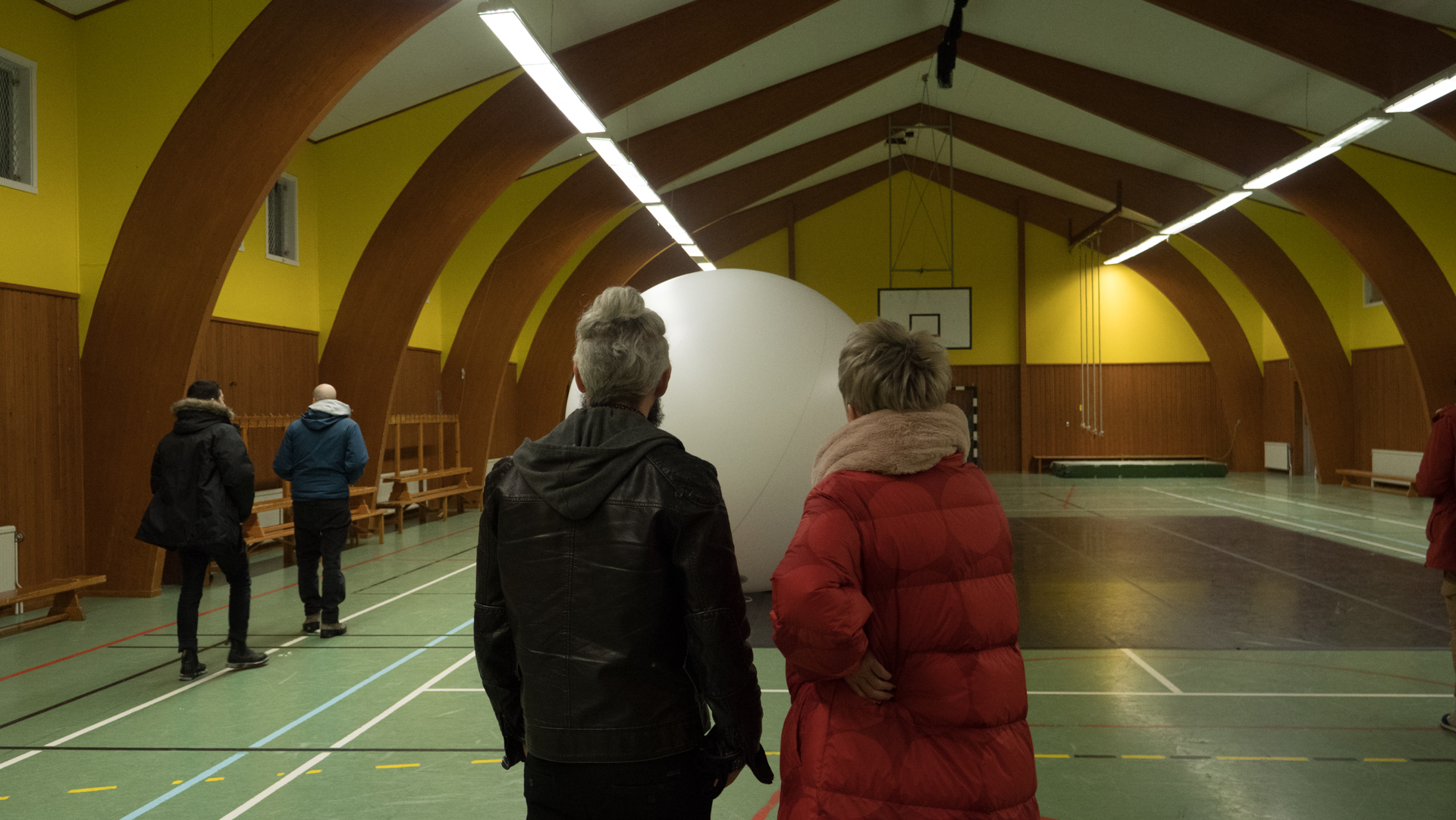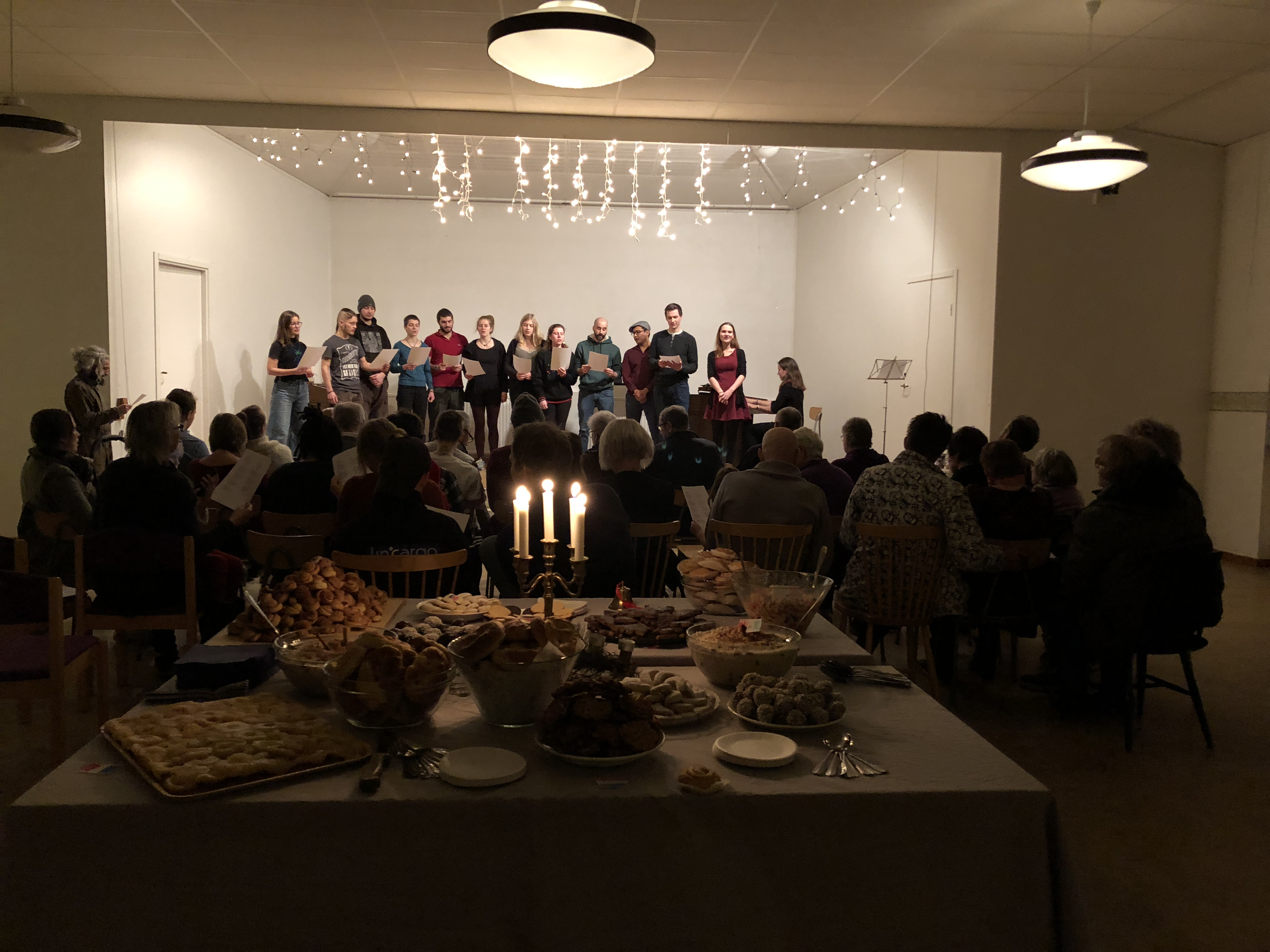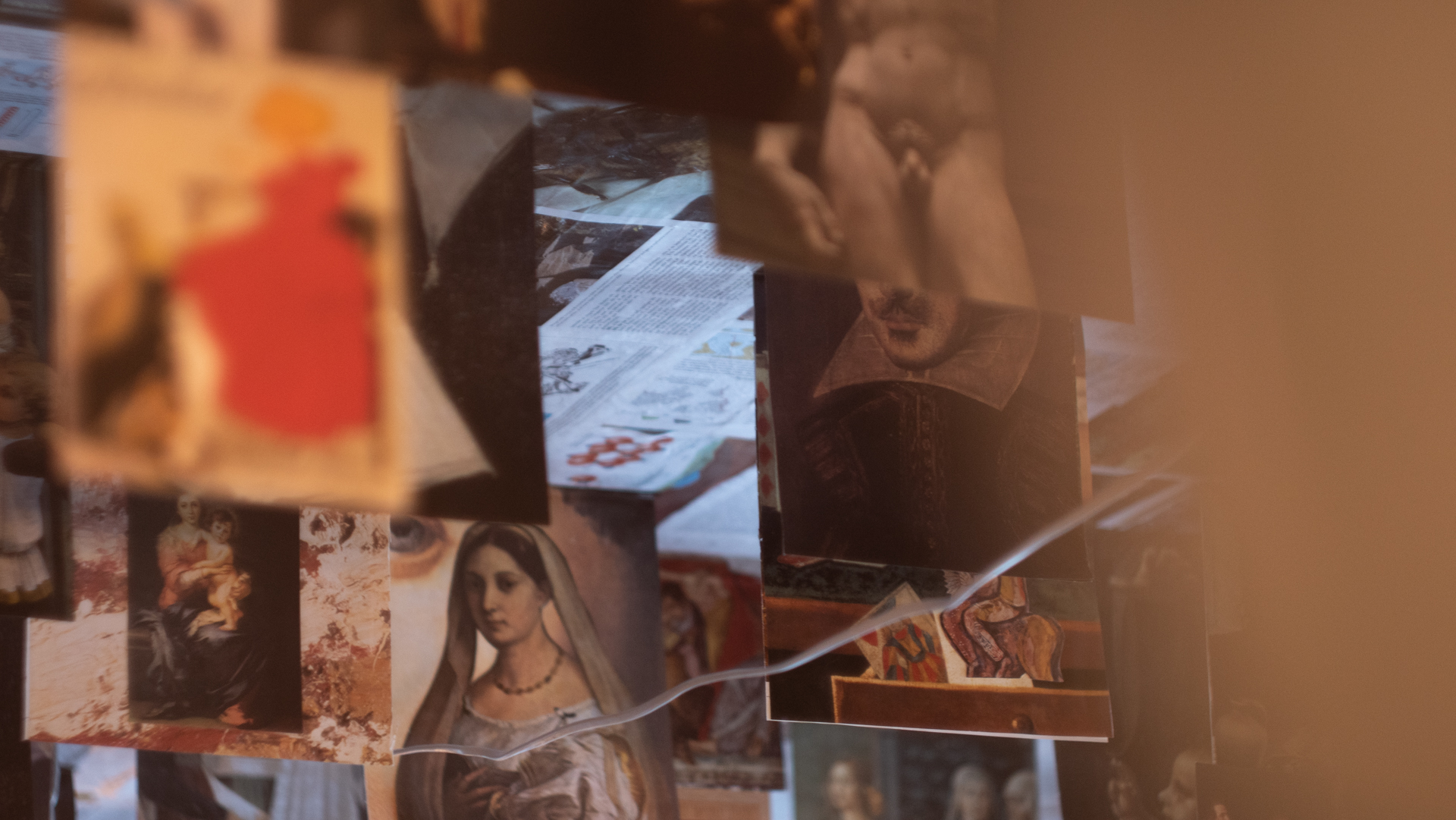# START MAKING

## Ongoing,

###### info@northernsustainablefutures.com

<style>
canvas {
position: absolute;
left:0;
top: 0;
width: 100%;
height: 100%;
background-color: rgba(0,0,0,.2);
}
</style>

<canvas id="heart"></canvas>

<script>
window.requestAnimationFrame =
window.__requestAnimationFrame ||
window.requestAnimationFrame ||
window.webkitRequestAnimationFrame ||
window.mozRequestAnimationFrame ||
window.oRequestAnimationFrame ||
window.msRequestAnimationFrame ||
(function () {
return function (callback, element) {
var lastTime = element.__lastTime;
if (lastTime === undefined) {
lastTime = 0;
}
var currTime = Date.now();
var timeToCall = Math.max(1, 33 - (currTime - lastTime));
window.setTimeout(callback, timeToCall);
element.__lastTime = currTime + timeToCall;
};
})();
window.isDevice = (/android|webos|iphone|ipad|ipod|blackberry|iemobile|opera mini/i.test(((navigator.userAgent || navigator.vendor || window.opera)).toLowerCase()));
var init = function () {
var mobile = window.isDevice;
var koef = mobile ? 0.5 : 1;
var canvas = document.getElementById('heart');
var ctx = canvas.getContext('2d');
var width = canvas.width = koef * innerWidth;
var height = canvas.height = koef * innerHeight;
var rand = Math.random;
ctx.fillStyle = "rgba(0,0,0,1)";
ctx.fillRect(0, 0, width, height);

var heartPosition = function (rad) {
};
var scaleAndTranslate = function (pos, sx, sy, dx, dy) {
return [dx + pos * sx, dy + pos * sy];
};

width = canvas.width = koef * innerWidth;
height = canvas.height = koef * innerHeight;
ctx.fillStyle = "rgba(0,0,0,1)";
ctx.fillRect(0, 0, width, height);
});

var traceCount = mobile ? 20 : 50;
var pointsOrigin = [];
var i;
var dr = mobile ? 0.3 : 0.1;
for (i = 0; i < Math.PI * 2; i += dr) pointsOrigin.push(scaleAndTranslate(heartPosition(i), 210, 13, 0, 0));
for (i = 0; i < Math.PI * 2; i += dr) pointsOrigin.push(scaleAndTranslate(heartPosition(i), 150, 9, 0, 0));
for (i = 0; i < Math.PI * 2; i += dr) pointsOrigin.push(scaleAndTranslate(heartPosition(i), 90, 5, 0, 0));
var heartPointsCount = pointsOrigin.length;

var targetPoints = [];
var pulse = function (kx, ky) {
for (i = 0; i < pointsOrigin.length; i++) {
targetPoints[i] = [];
targetPoints[i] = kx * pointsOrigin[i] + width / 2;
targetPoints[i] = ky * pointsOrigin[i] + height / 2;
}
};

var e = [];
for (i = 0; i < heartPointsCount; i++) {
var x = rand() * width;
var y = rand() * height;
e[i] = {
vx: 0,
vy: 0,
R: 2,
speed: rand() + 5,
q: ~~(rand() * heartPointsCount),
D: 2 * (i % 2) - 1,
force: 0.2 * rand() + 0.7,
f: "hsla(0," + ~~(40 * rand() + 60) + "%," + ~~(60 * rand() + 20) + "%,.3)",
trace: []
};
for (var k = 0; k < traceCount; k++) e[i].trace[k] = {x: x, y: y};
}

var config = {
traceK: 0.4,
timeDelta: 0.01
};

var time = 0;
var loop = function () {
var n = -Math.cos(time);
pulse((1 + n) * .5, (1 + n) * .5);
time += ((Math.sin(time)) < 0 ? 9 : (n > 0.8) ? .2 : 1) * config.timeDelta;
ctx.fillStyle = "rgba(0,0,0,.1)";
ctx.fillRect(0, 0, width, height);
for (i = e.length; i--;) {
var u = e[i];
var q = targetPoints[u.q];
var dx = u.trace.x - q;
var dy = u.trace.y - q;
var length = Math.sqrt(dx * dx + dy * dy);
if (10 > length) {
if (0.95 < rand()) {
u.q = ~~(rand() * heartPointsCount);
}
else {
if (0.99 < rand()) {
u.D *= -1;
}
u.q += u.D;
u.q %= heartPointsCount;
if (0 > u.q) {
u.q += heartPointsCount;
}
}
}
u.vx += -dx / length * u.speed;
u.vy += -dy / length * u.speed;
u.trace.x += u.vx;
u.trace.y += u.vy;
u.vx *= u.force;
u.vy *= u.force;
for (k = 0; k < u.trace.length - 1;) {
var T = u.trace[k];
var N = u.trace[++k];
N.x -= config.traceK * (N.x - T.x);
N.y -= config.traceK * (N.y - T.y);
}
ctx.fillStyle = u.f;
for (k = 0; k < u.trace.length; k++) {
ctx.fillRect(u.trace[k].x, u.trace[k].y, 1, 1);
}
}
//ctx.fillStyle = "rgba(255,255,255,1)";
//for (i = u.trace.length; i--;) ctx.fillRect(targetPoints[i], targetPoints[i], 2, 2);

window.requestAnimationFrame(loop, canvas);
};
loop();
};

if (s === 'complete' || s === 'loaded' || s === 'interactive') init();
</script>

The way we see the world creates the world we see.

Barry Neil Kaufman

Hover to see the hyper text markup language used to create the visual effect.

## MOSKOSEL CREATIVE LAB